# RS Aggarwal Solutions for Class 9 Chapter 1: Number Systems Exercise 1F

## RS Aggarwal Solutions for Class 9 Maths Exercise 1F PDF

The solutions are mainly prepared by our subject experts keeping in mind the syllabus followed in the RS Aggarwal textbook. In order to help students acquire a good hold on the concepts and topics in this chapter, the solutions are prepared in an explanatory manner. The students have to develop a habit of practising problems regularly to excel in a tough subject like Mathematics. RS Aggarwal Solutions mainly provides a huge number of examples before solving lengthy problems. RS Aggarwal Solutions for Class 9 Maths Chapter 1 Number Systems Exercise 1F are provided here.

## RS Aggarwal Solutions for Class 9 Chapter 1: Number Systems Exercise 1F Download PDF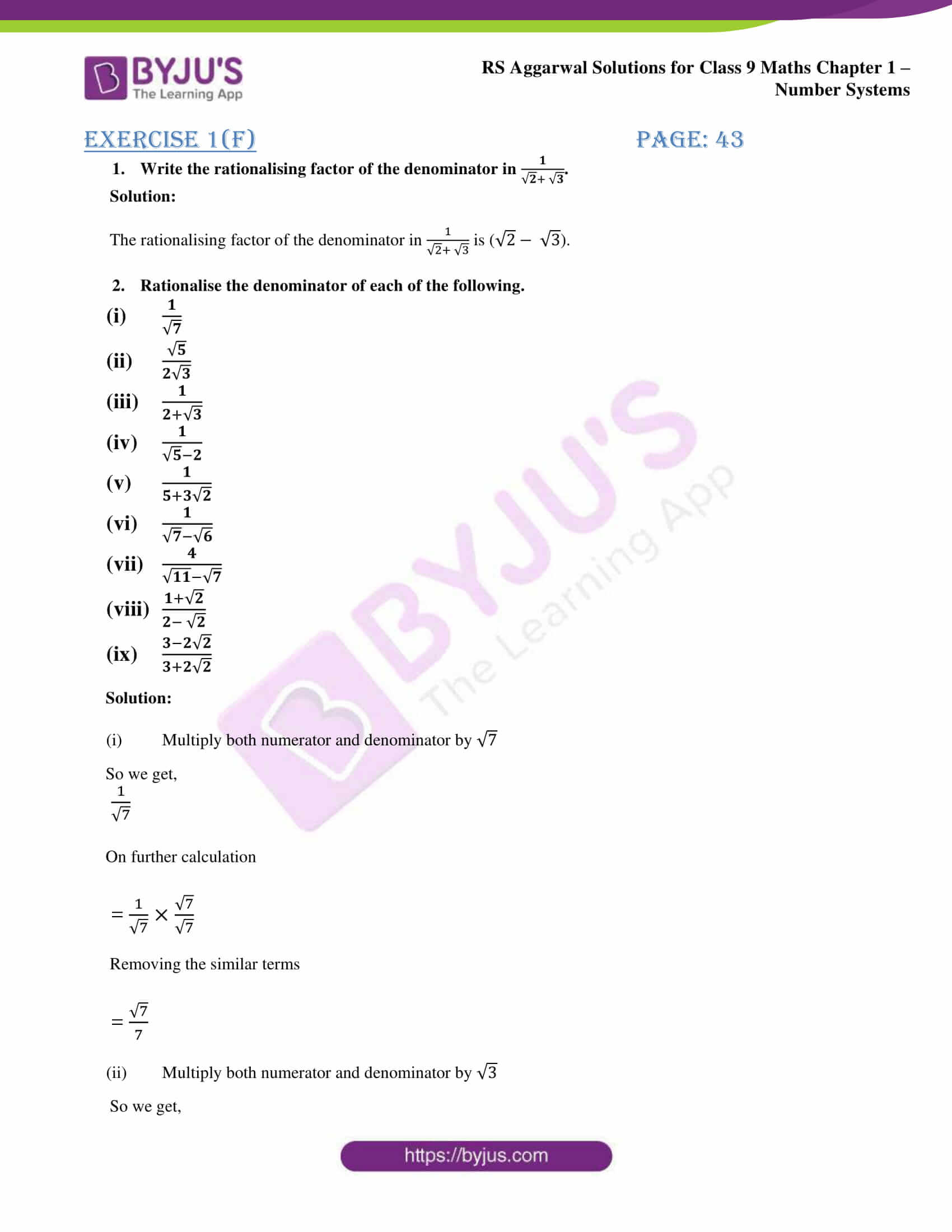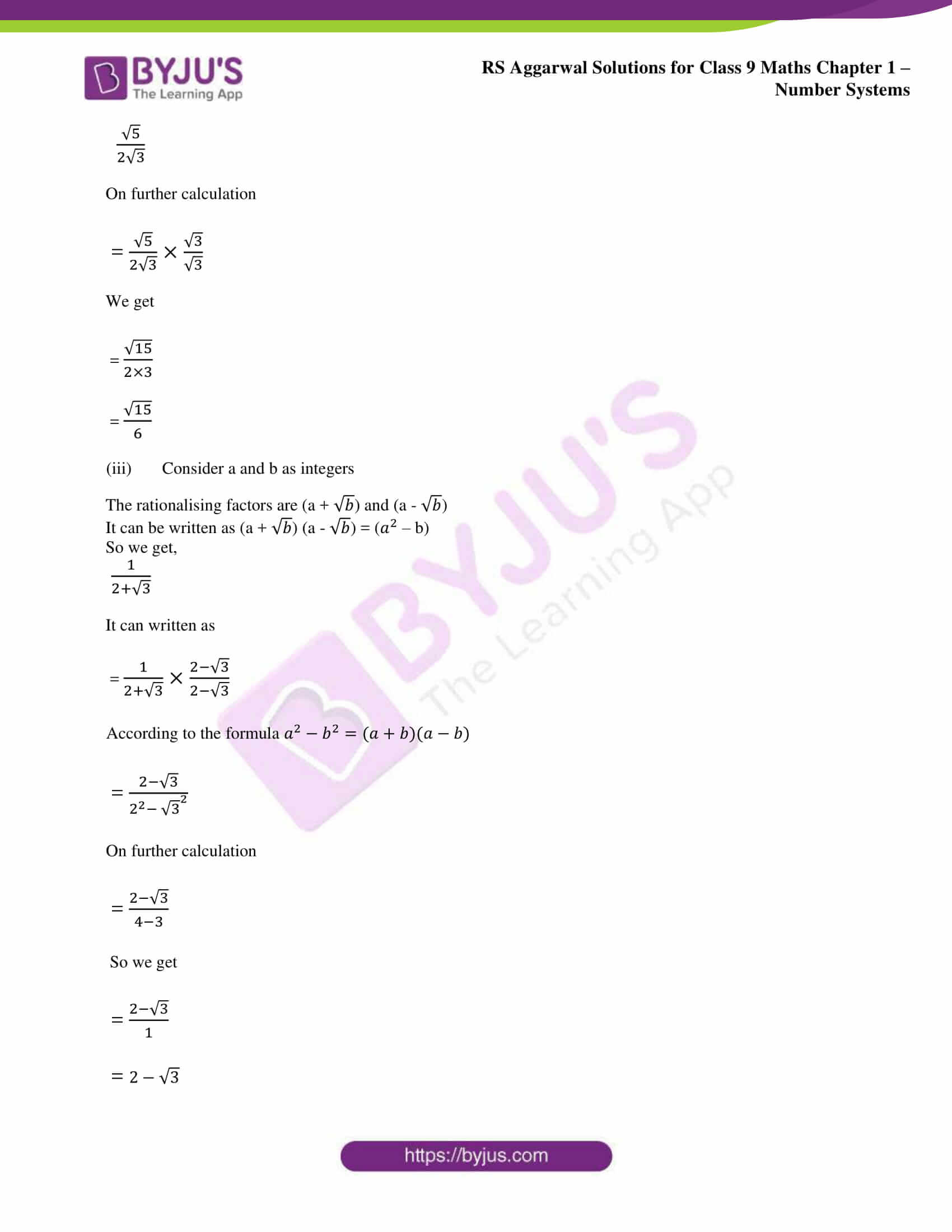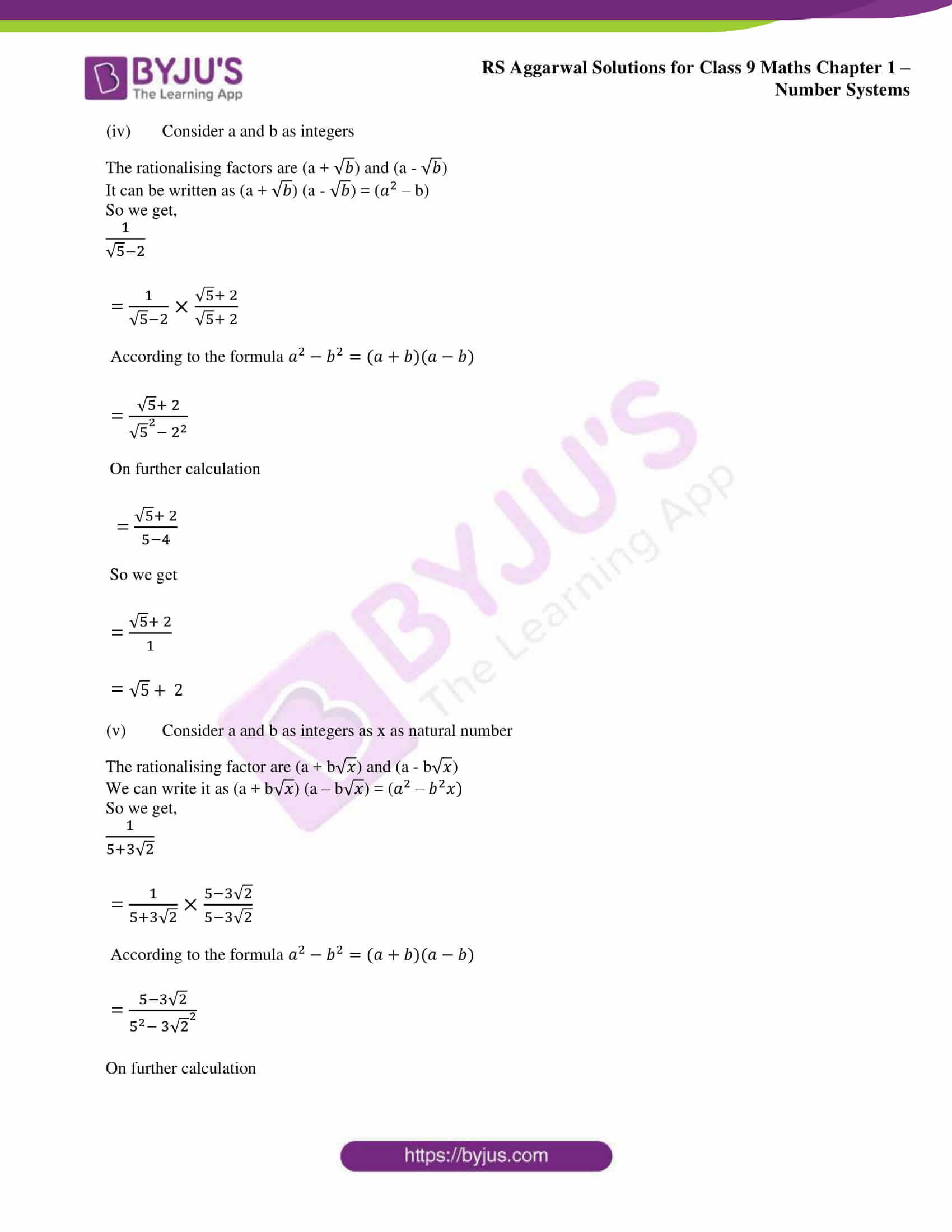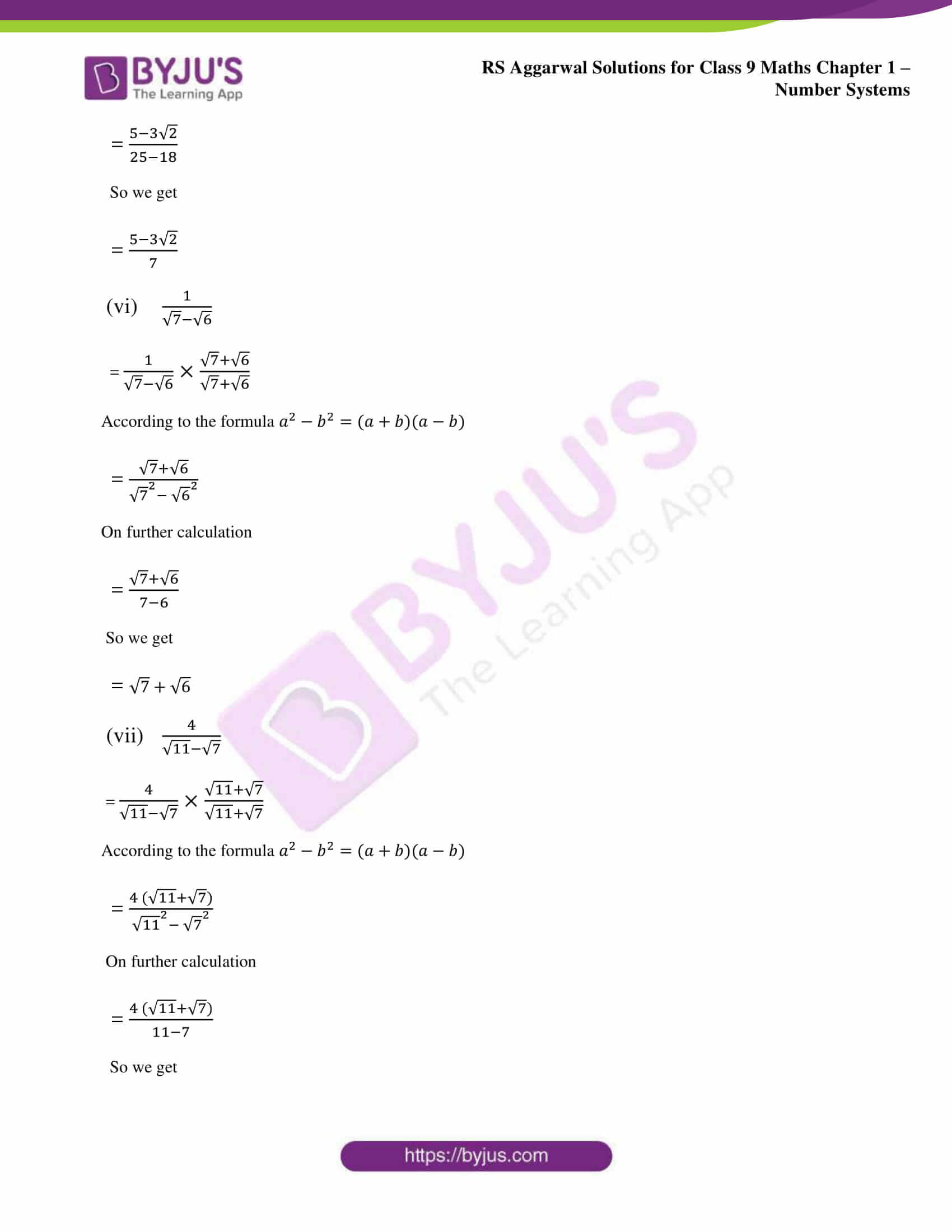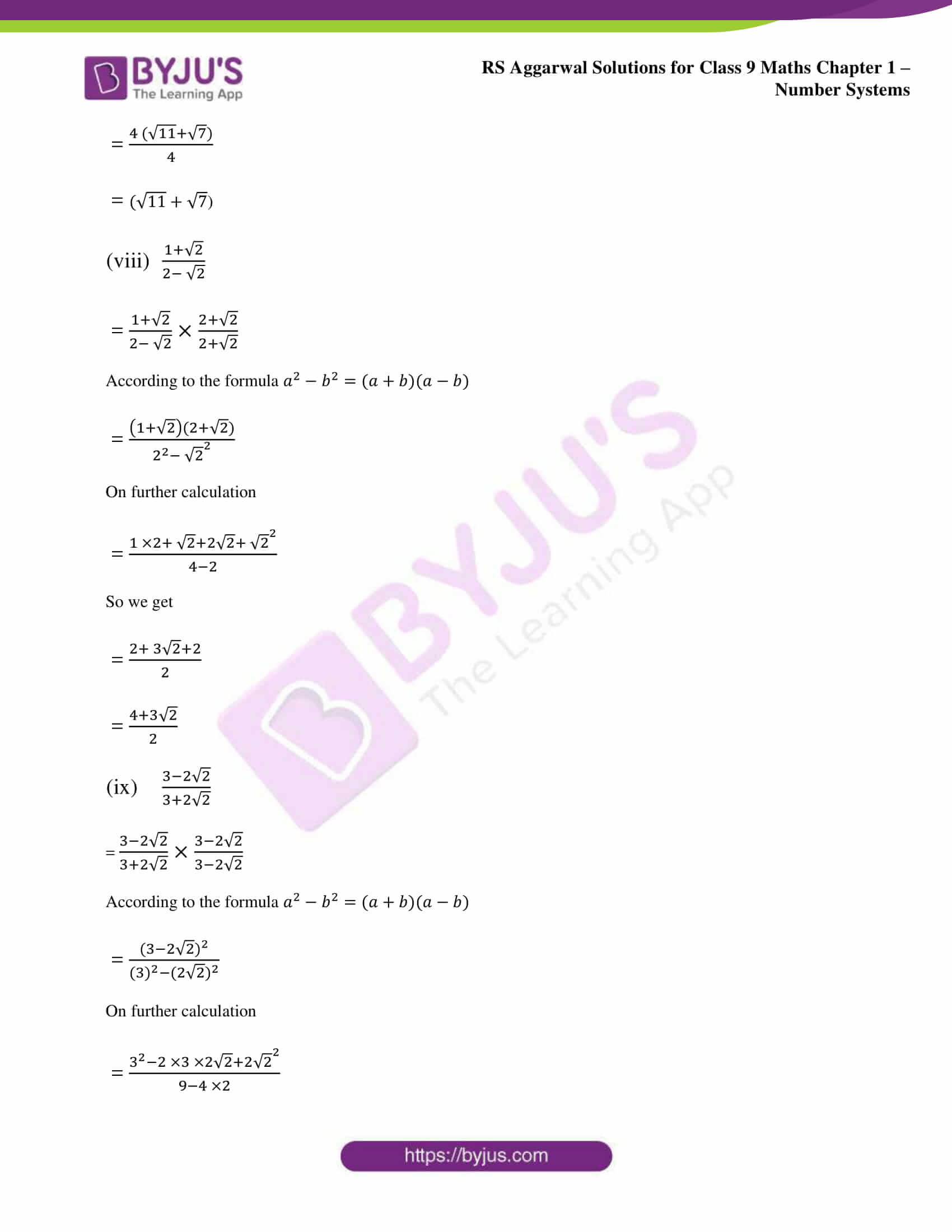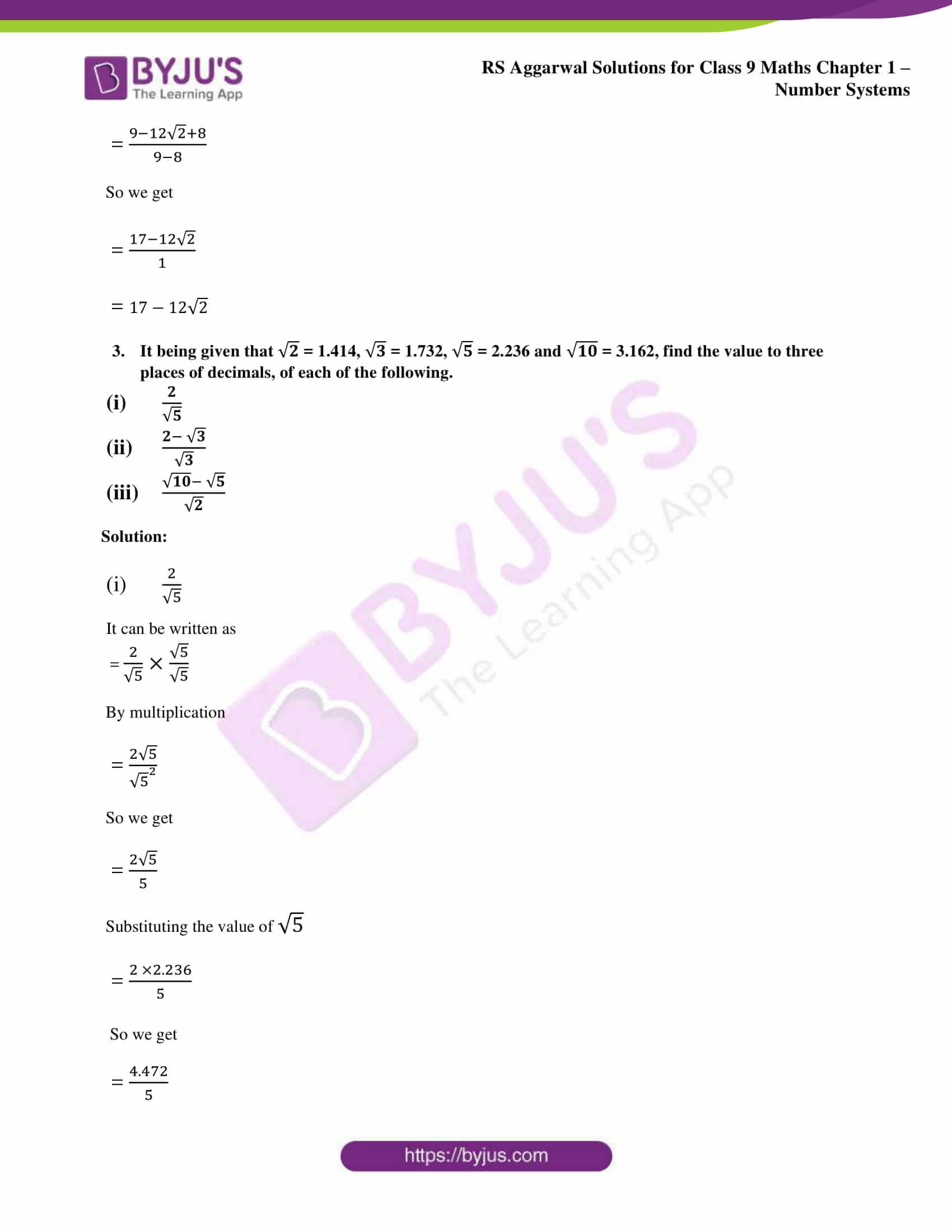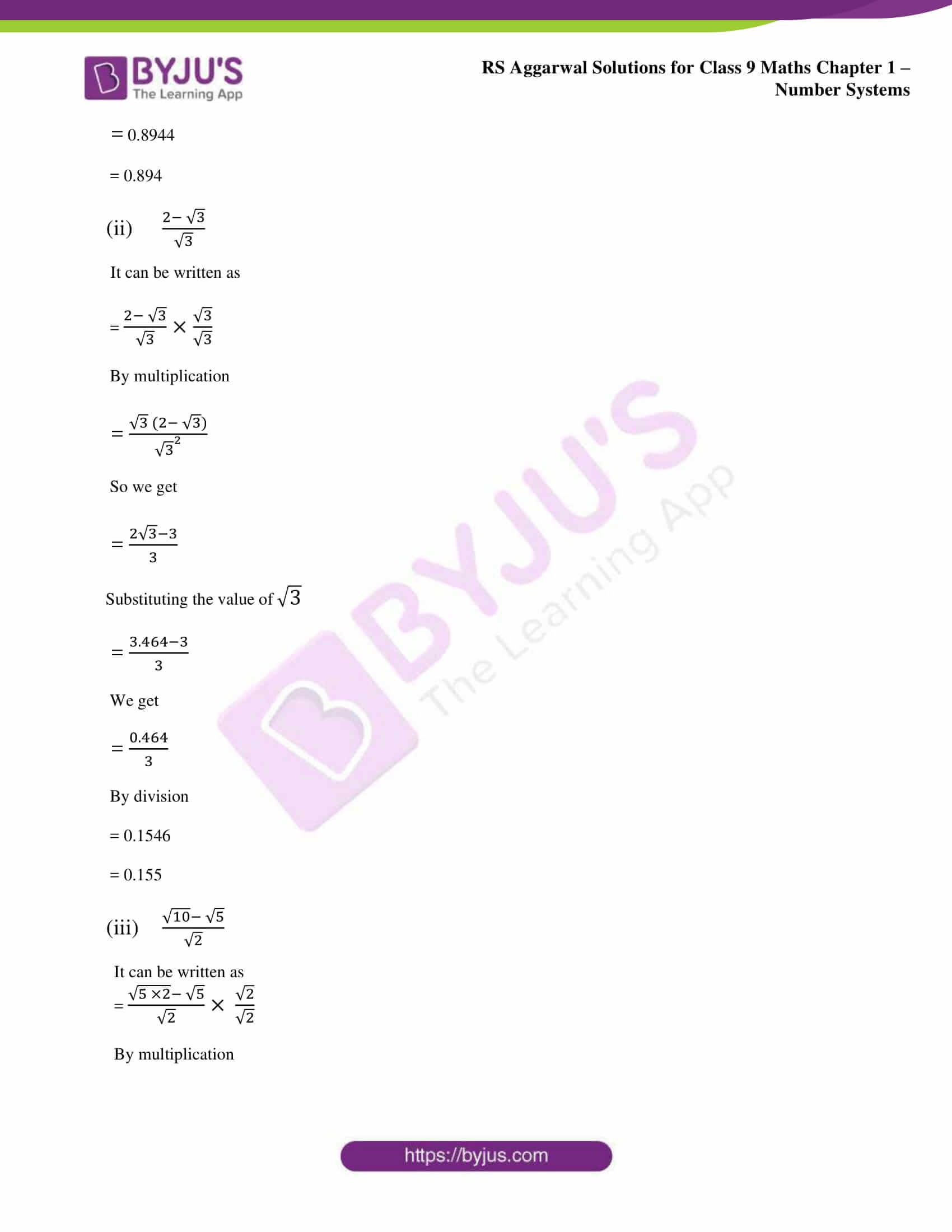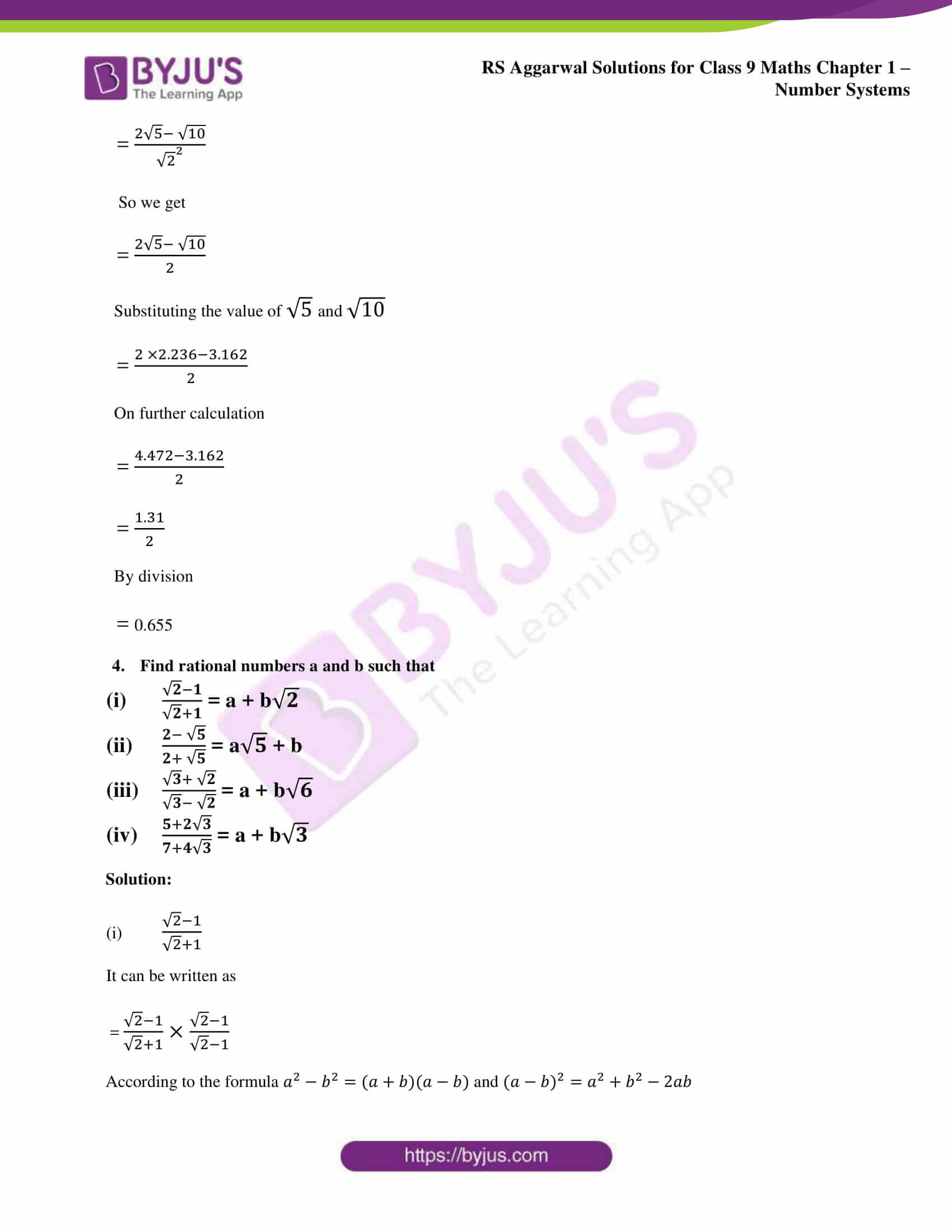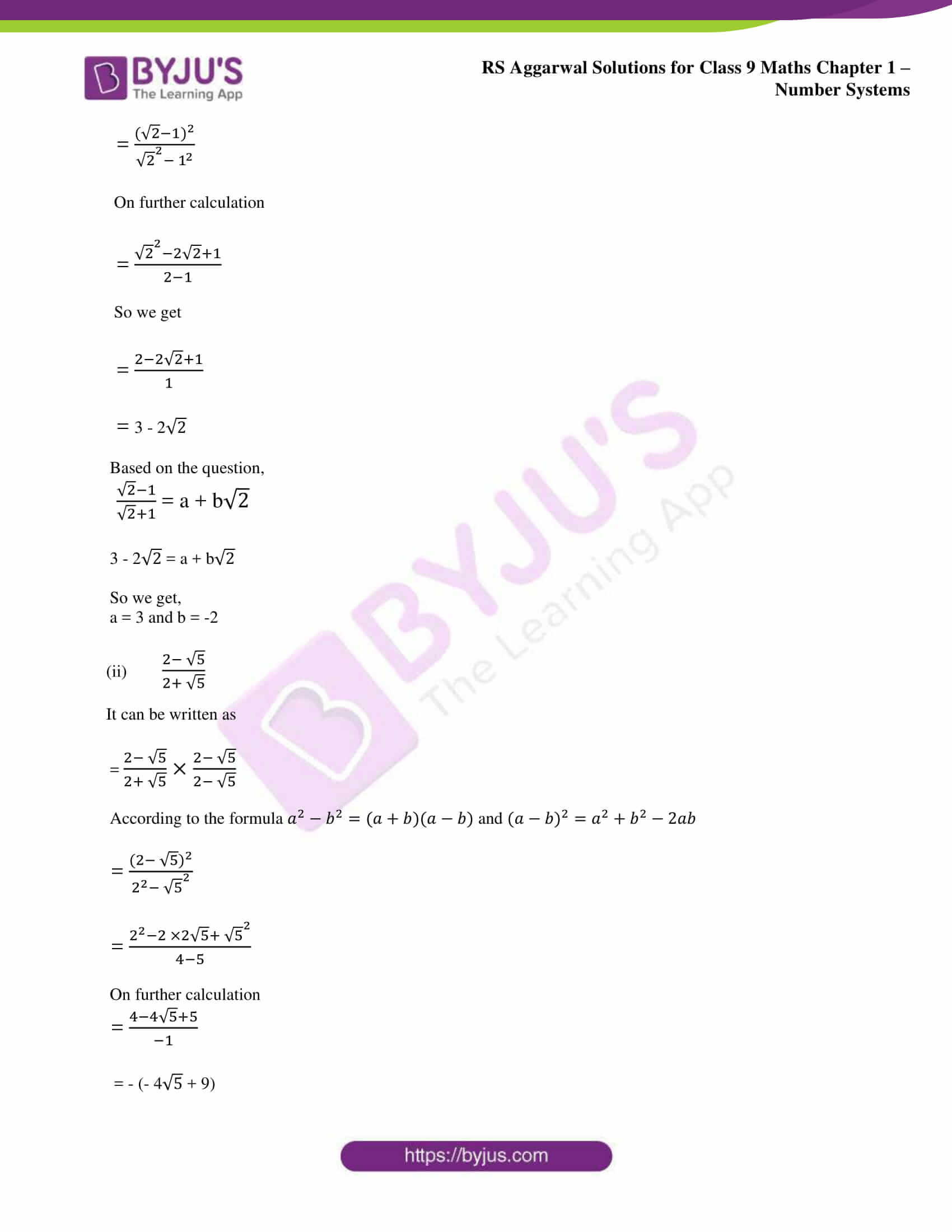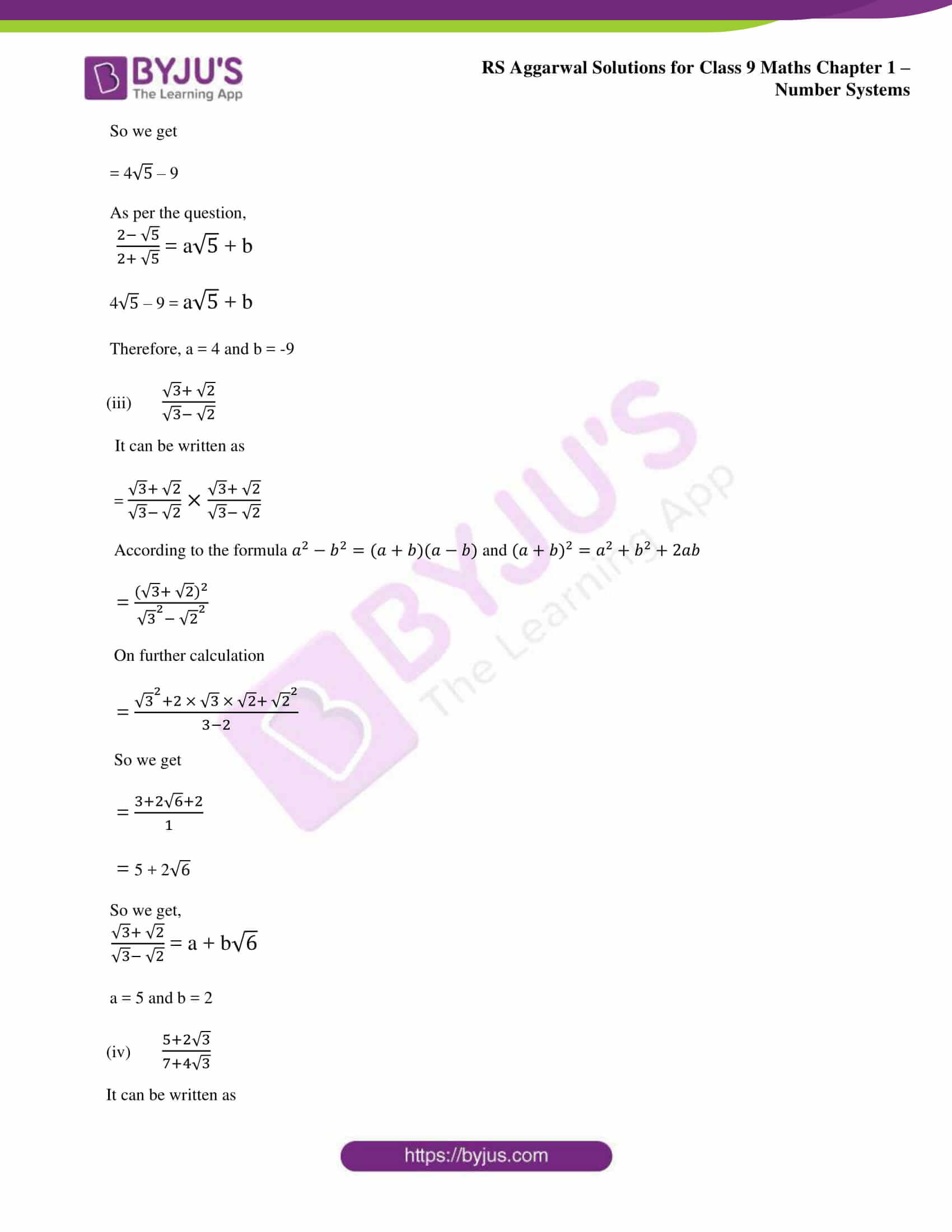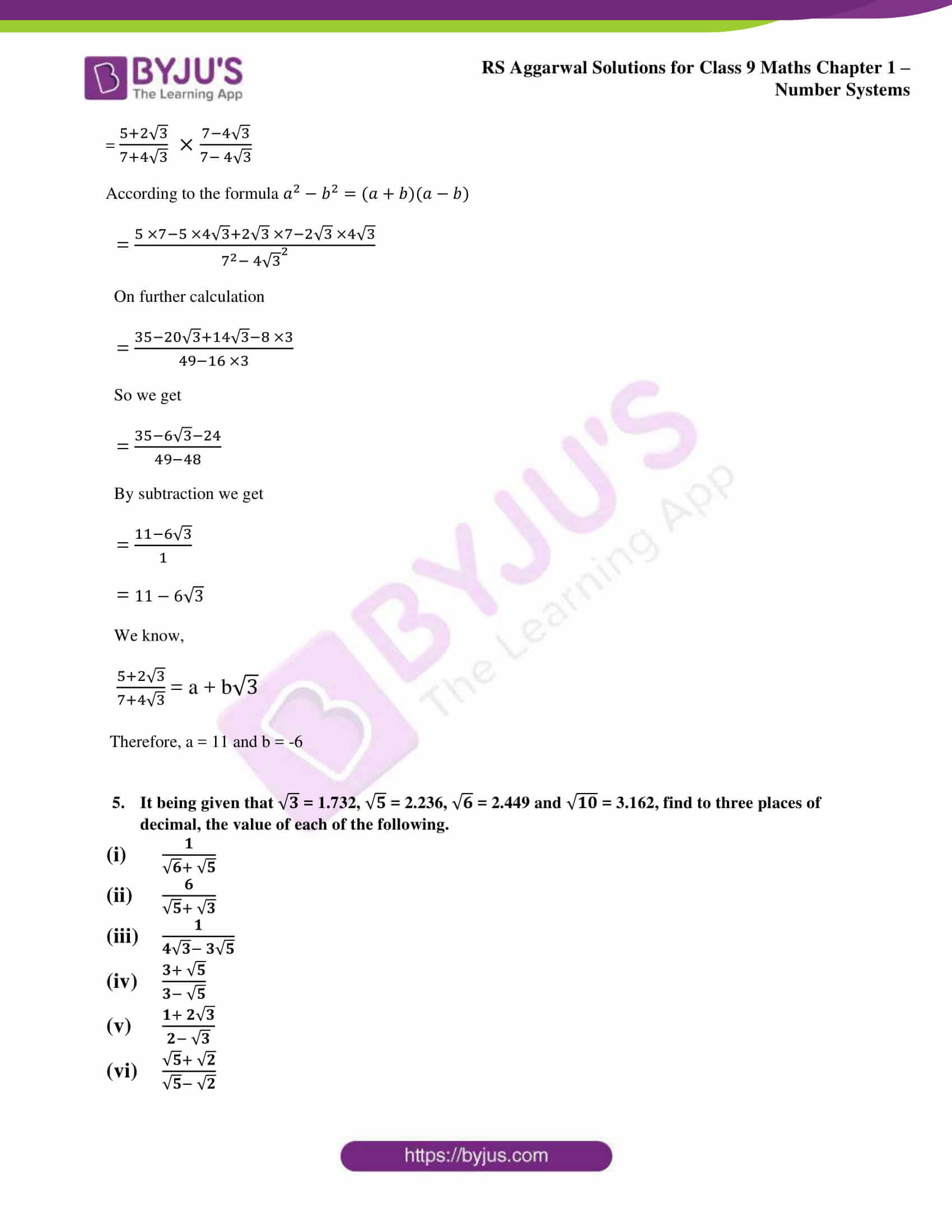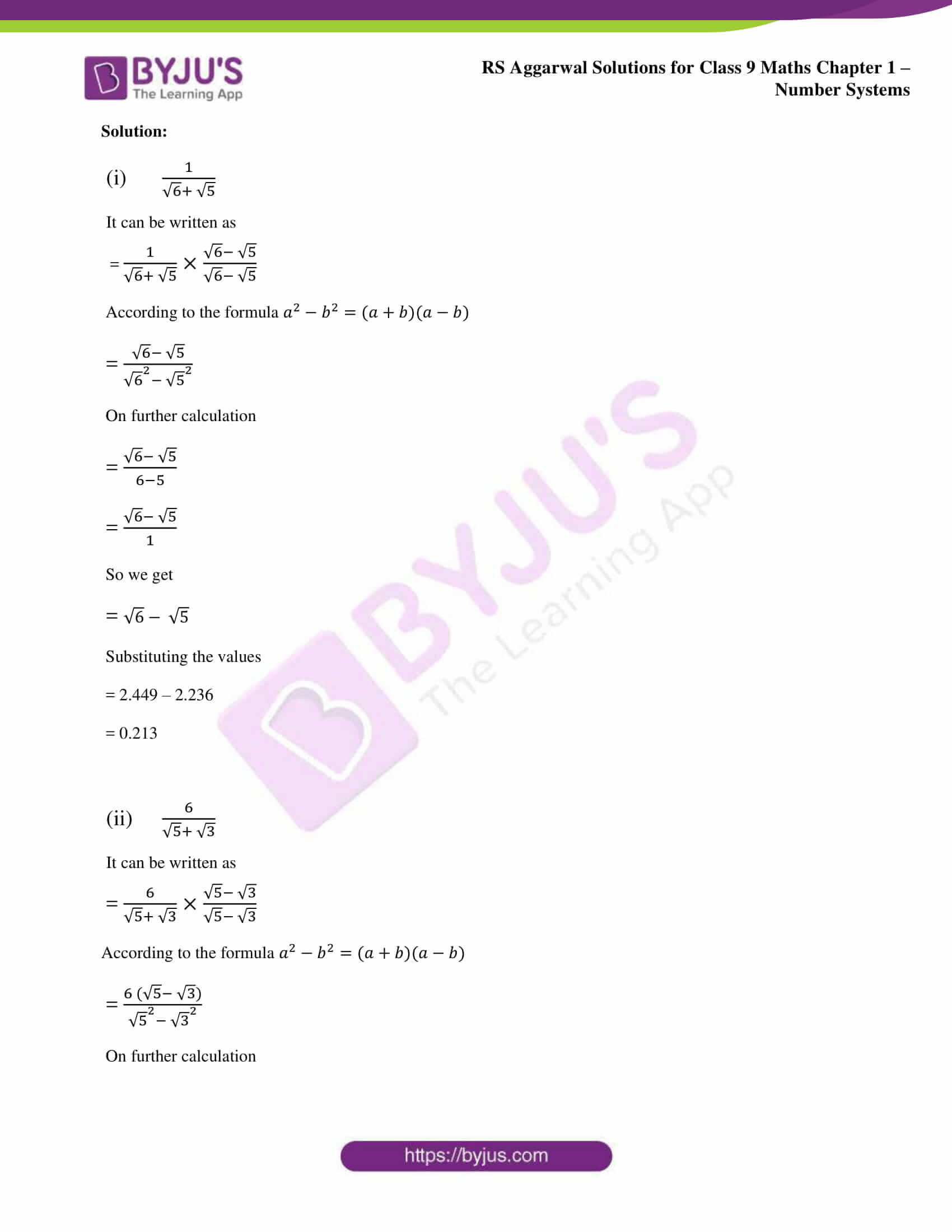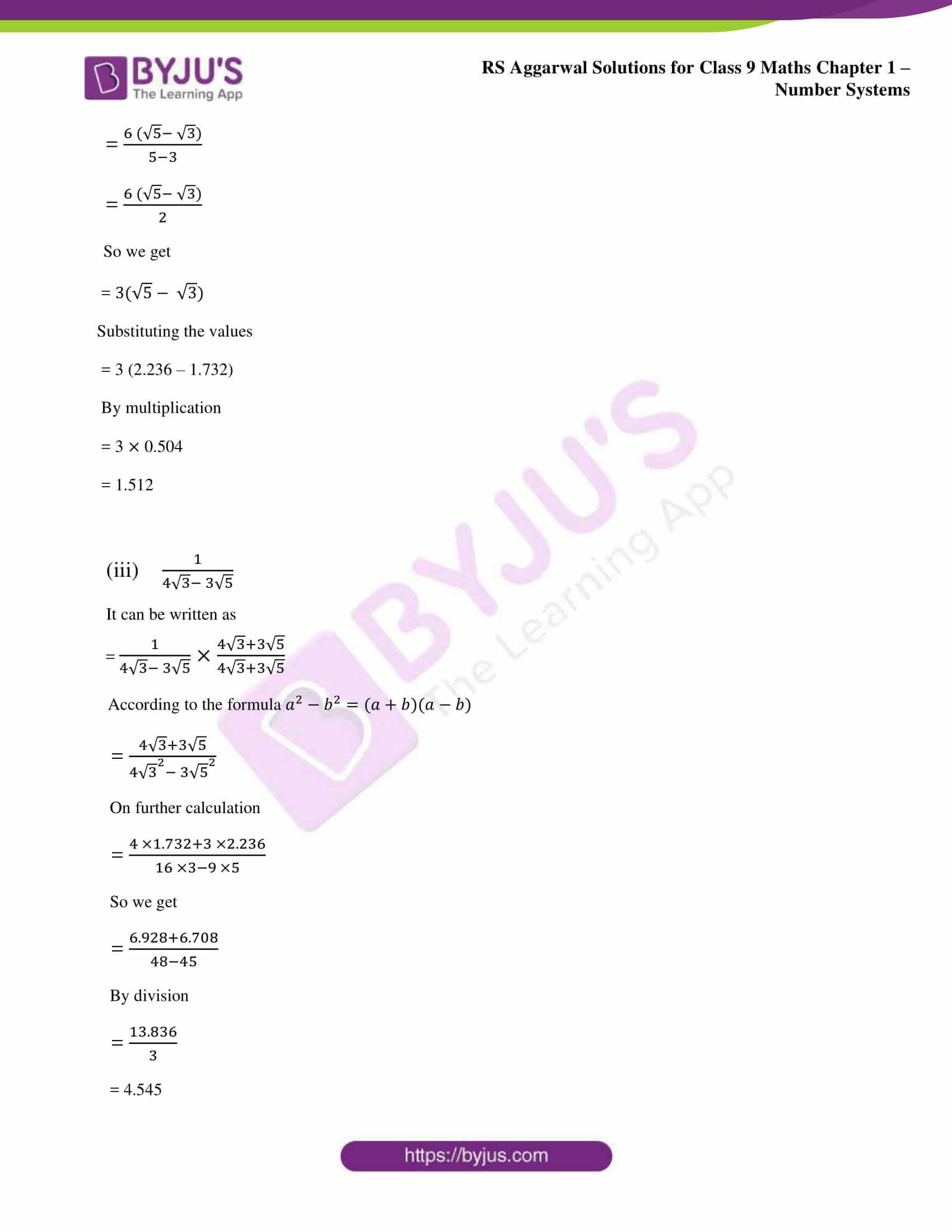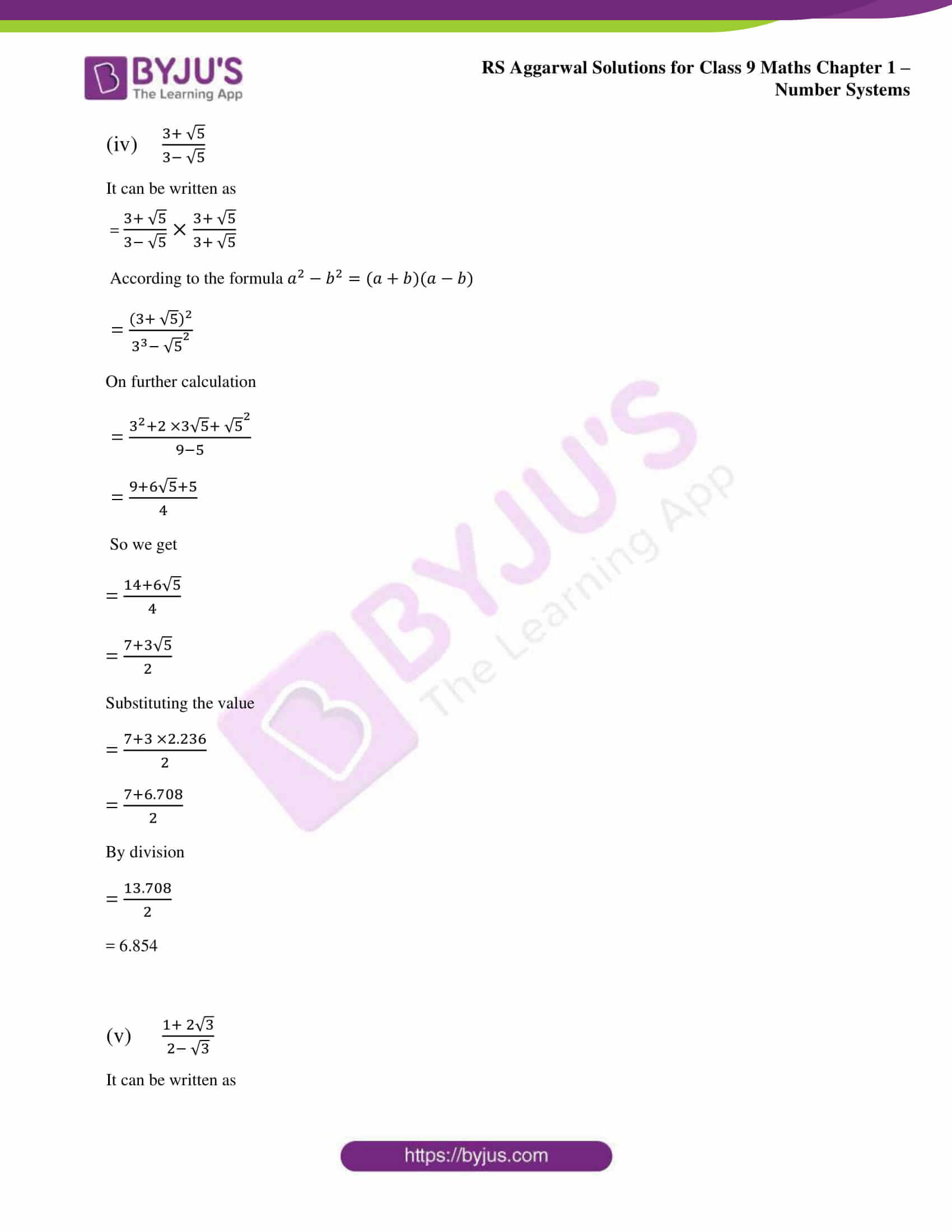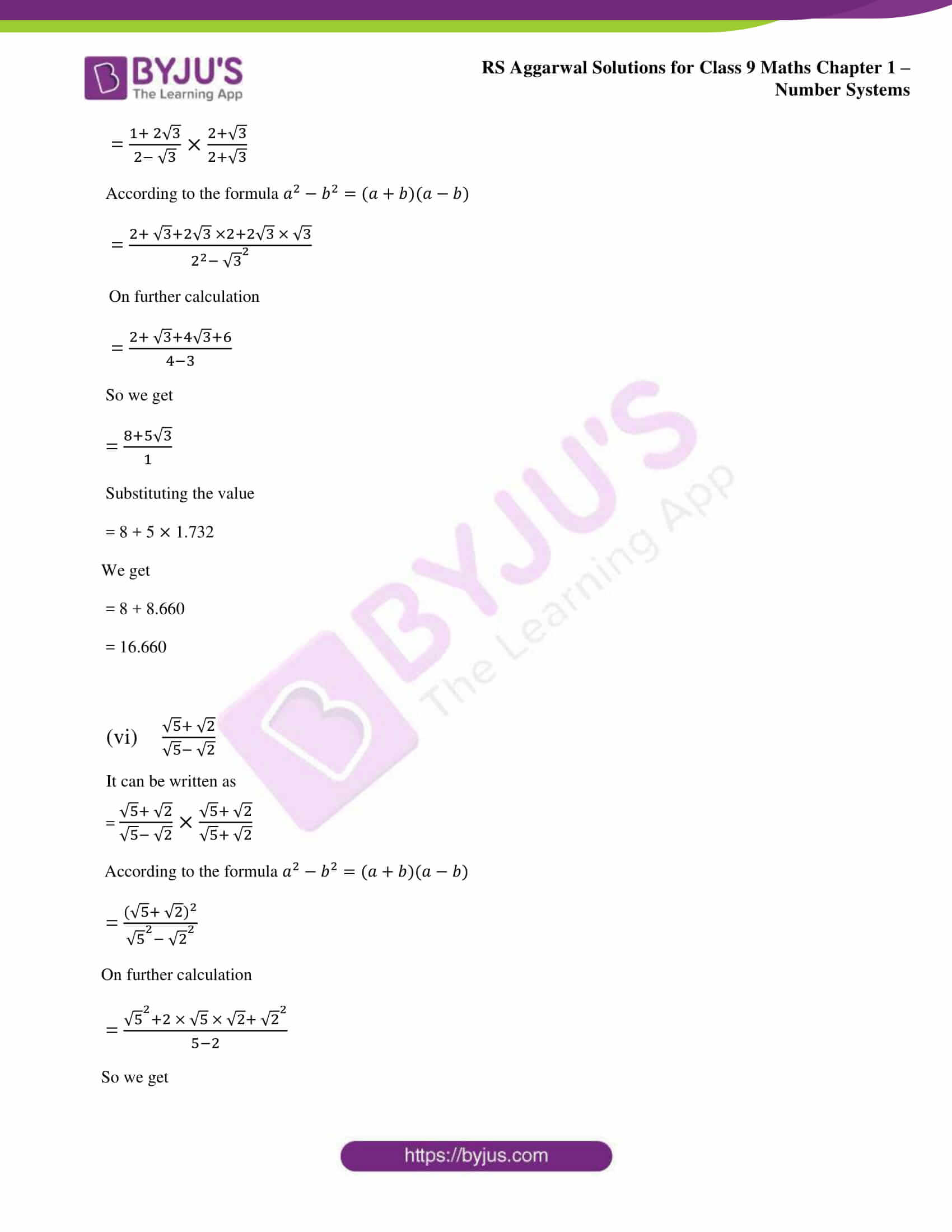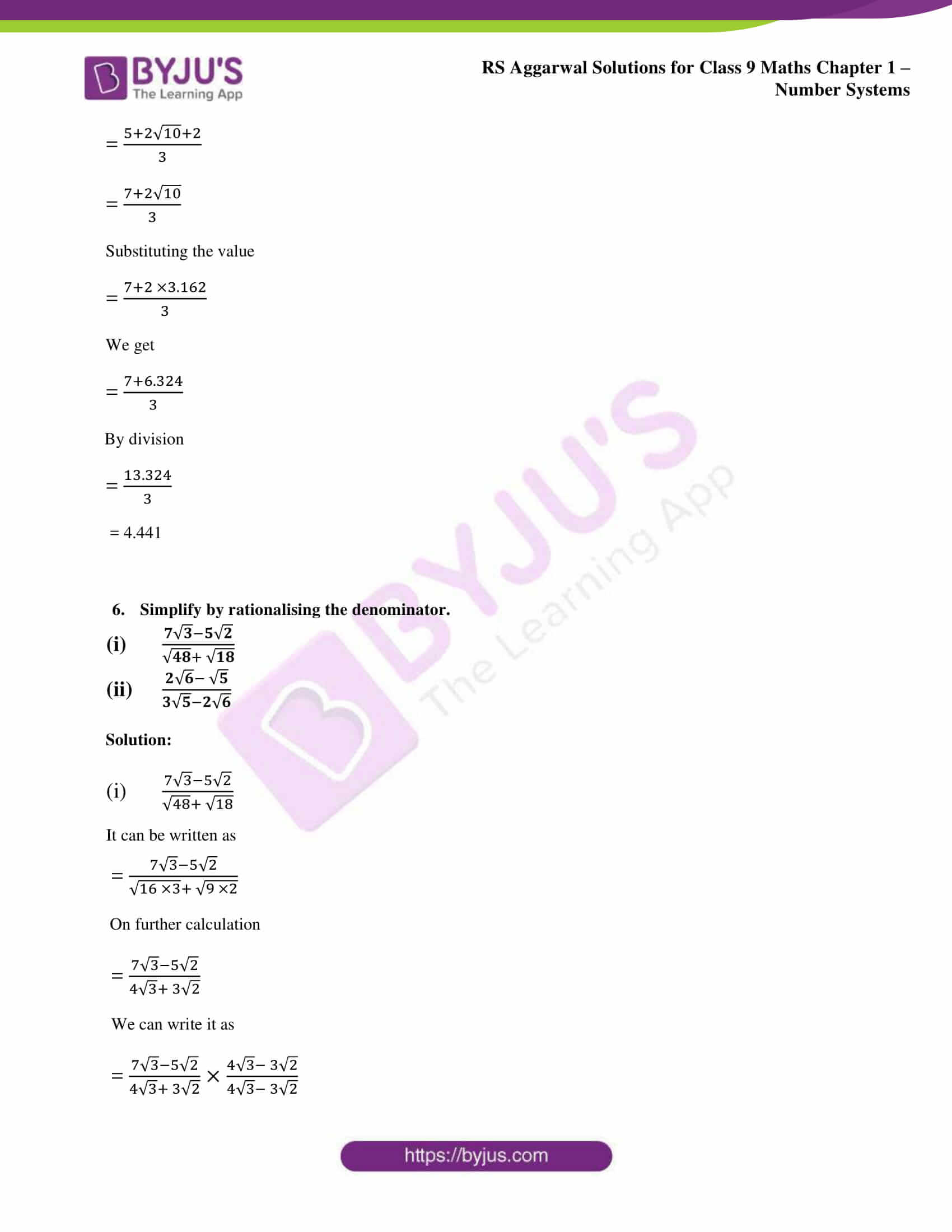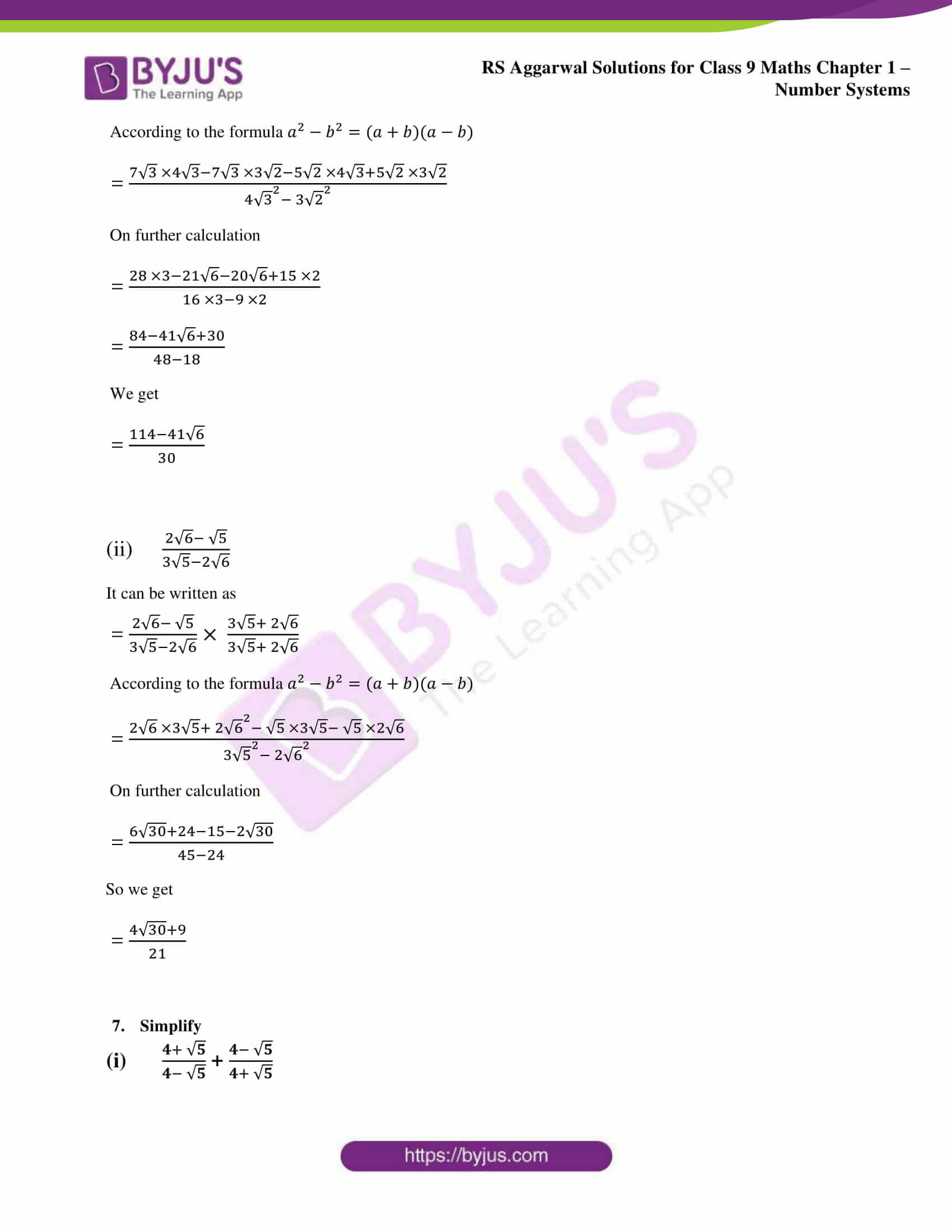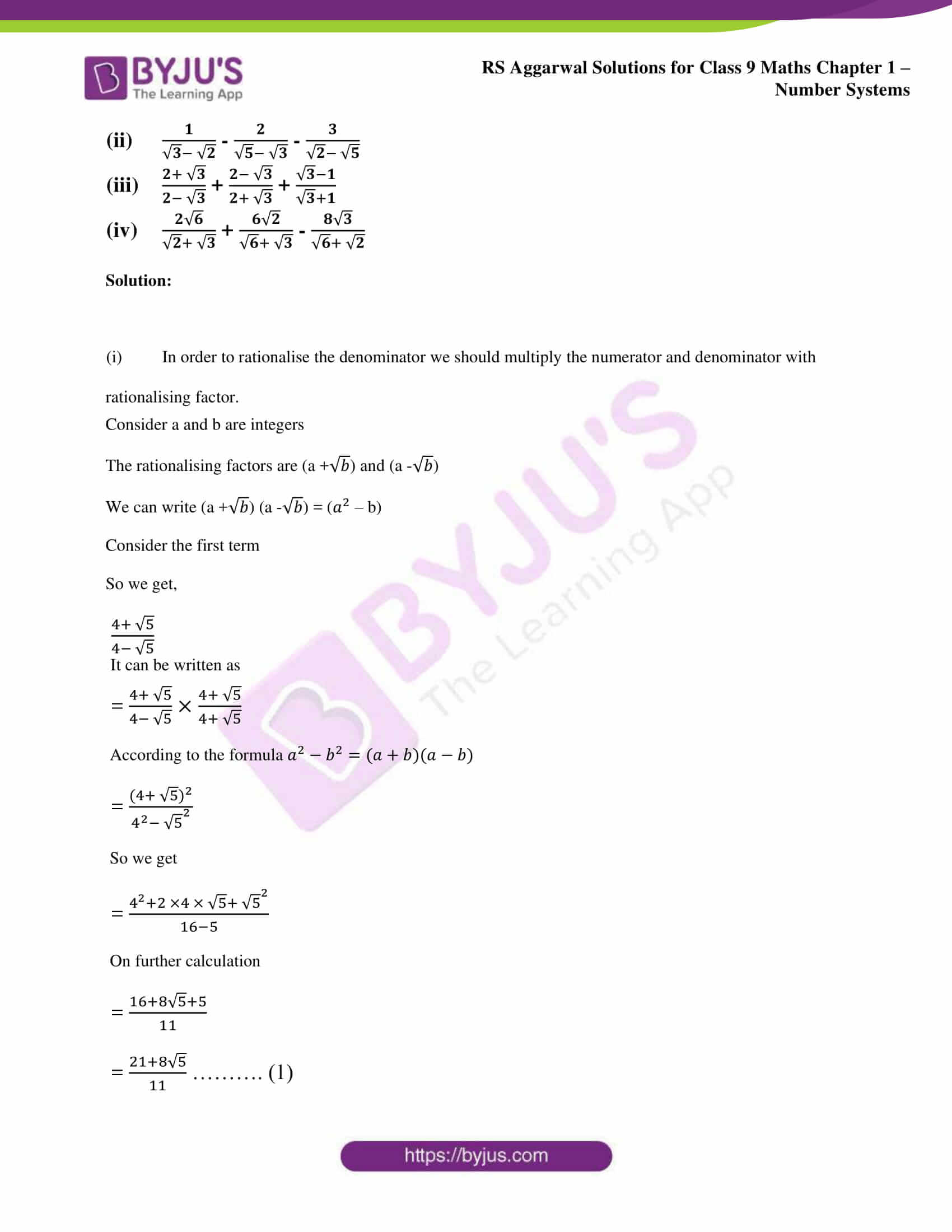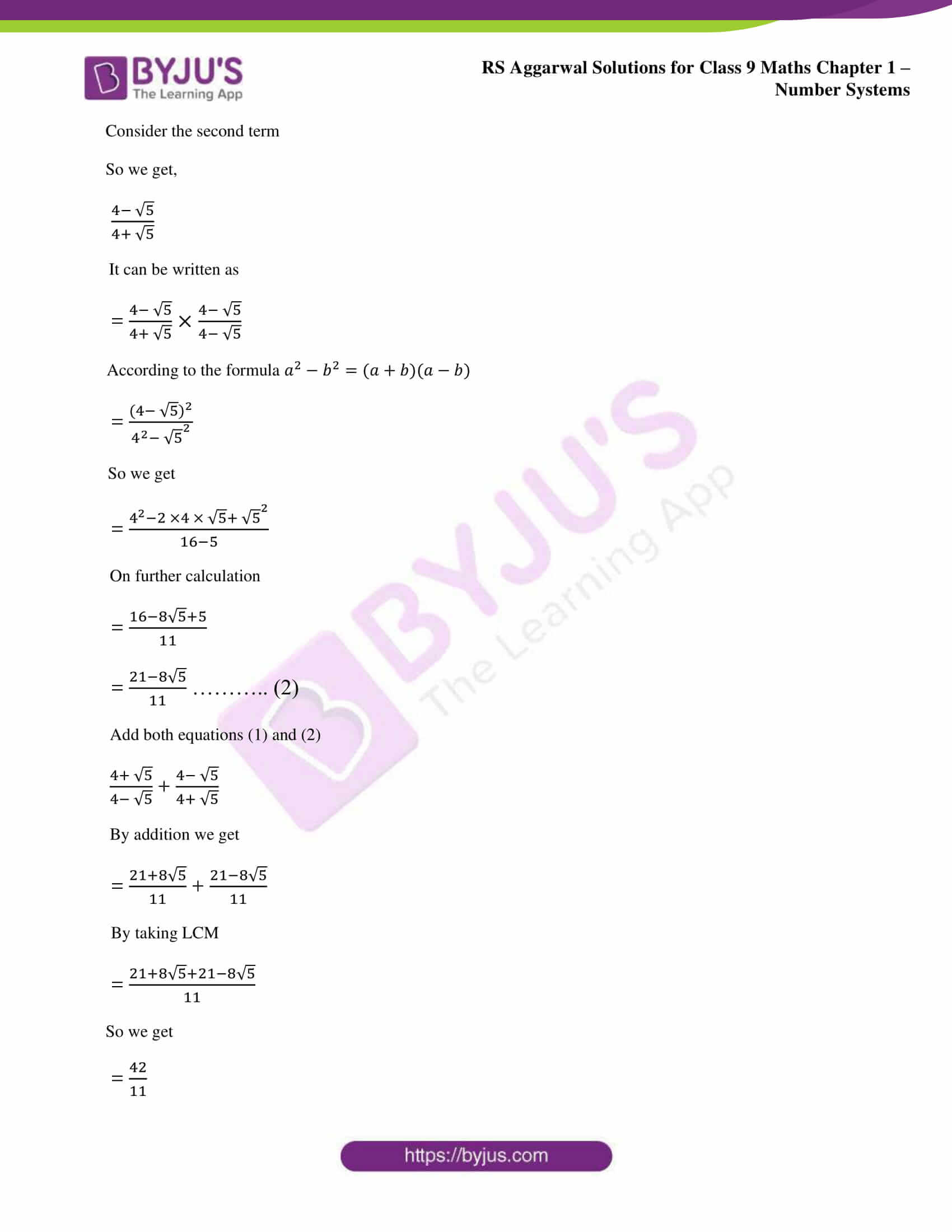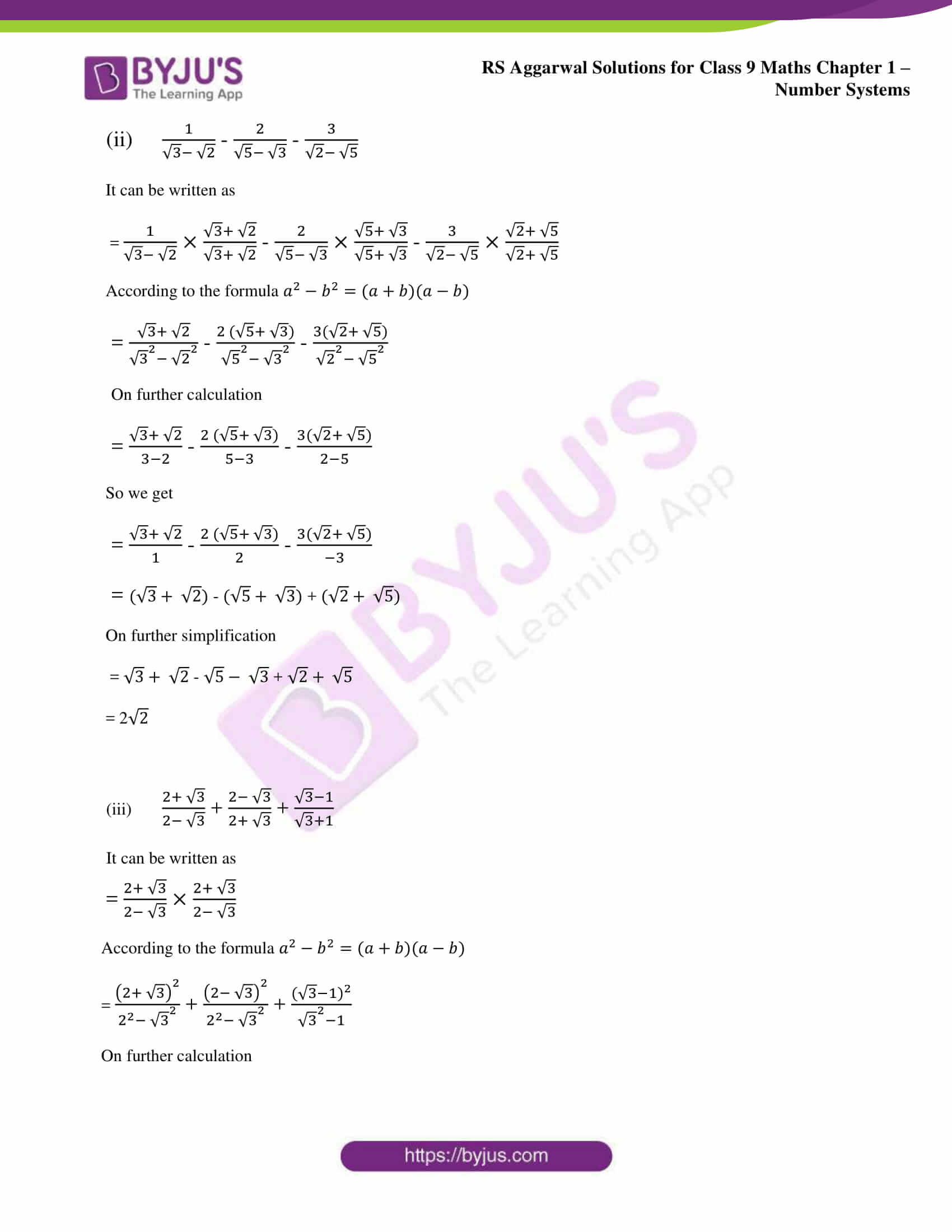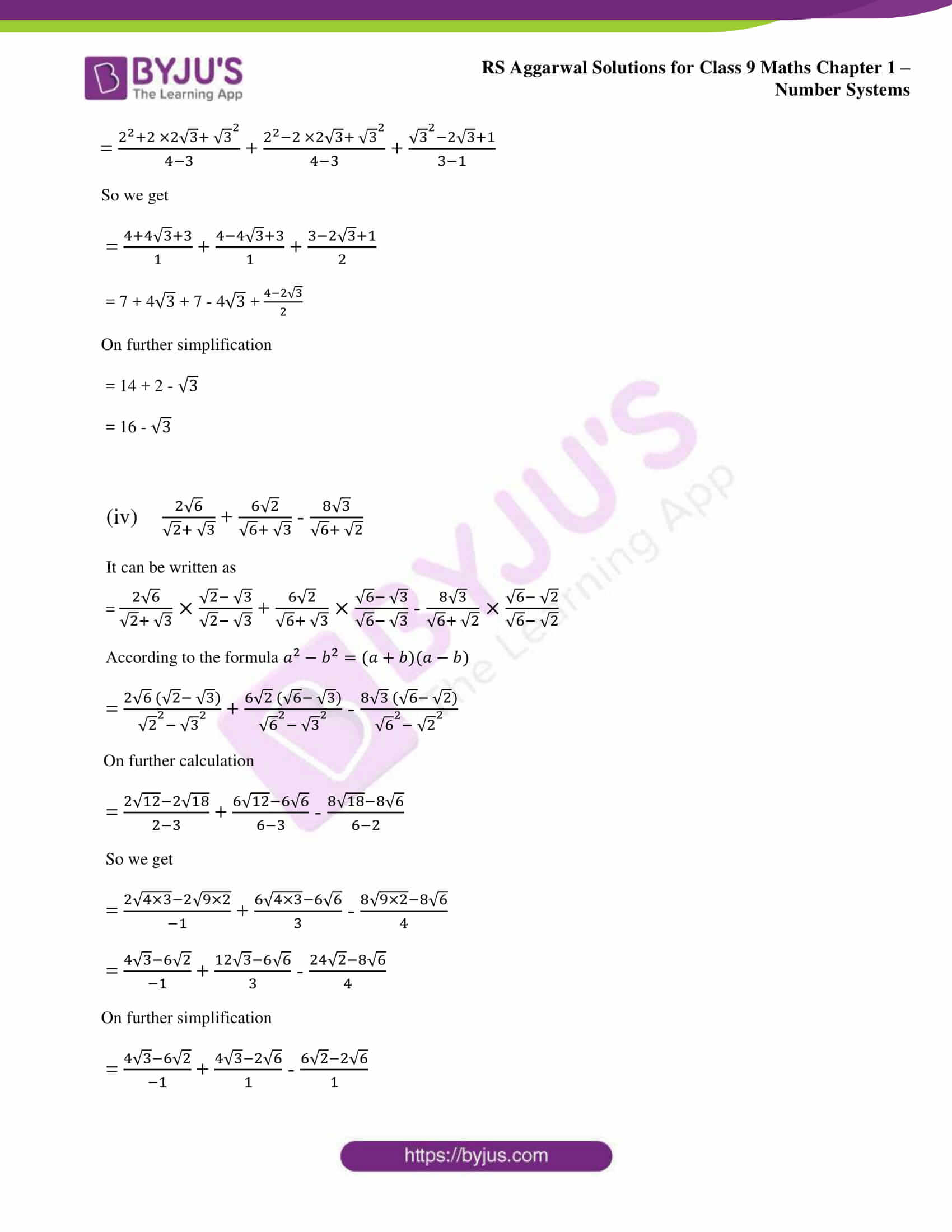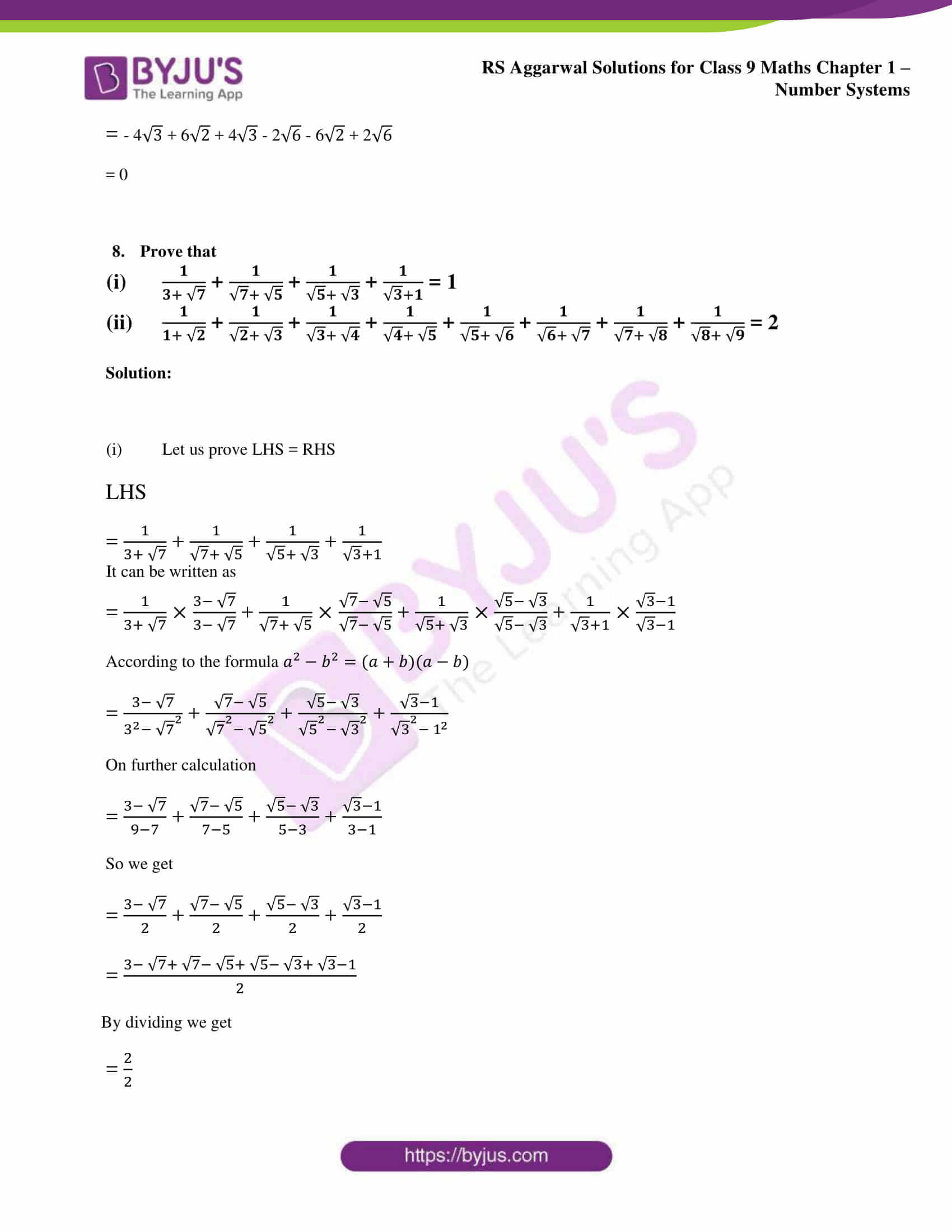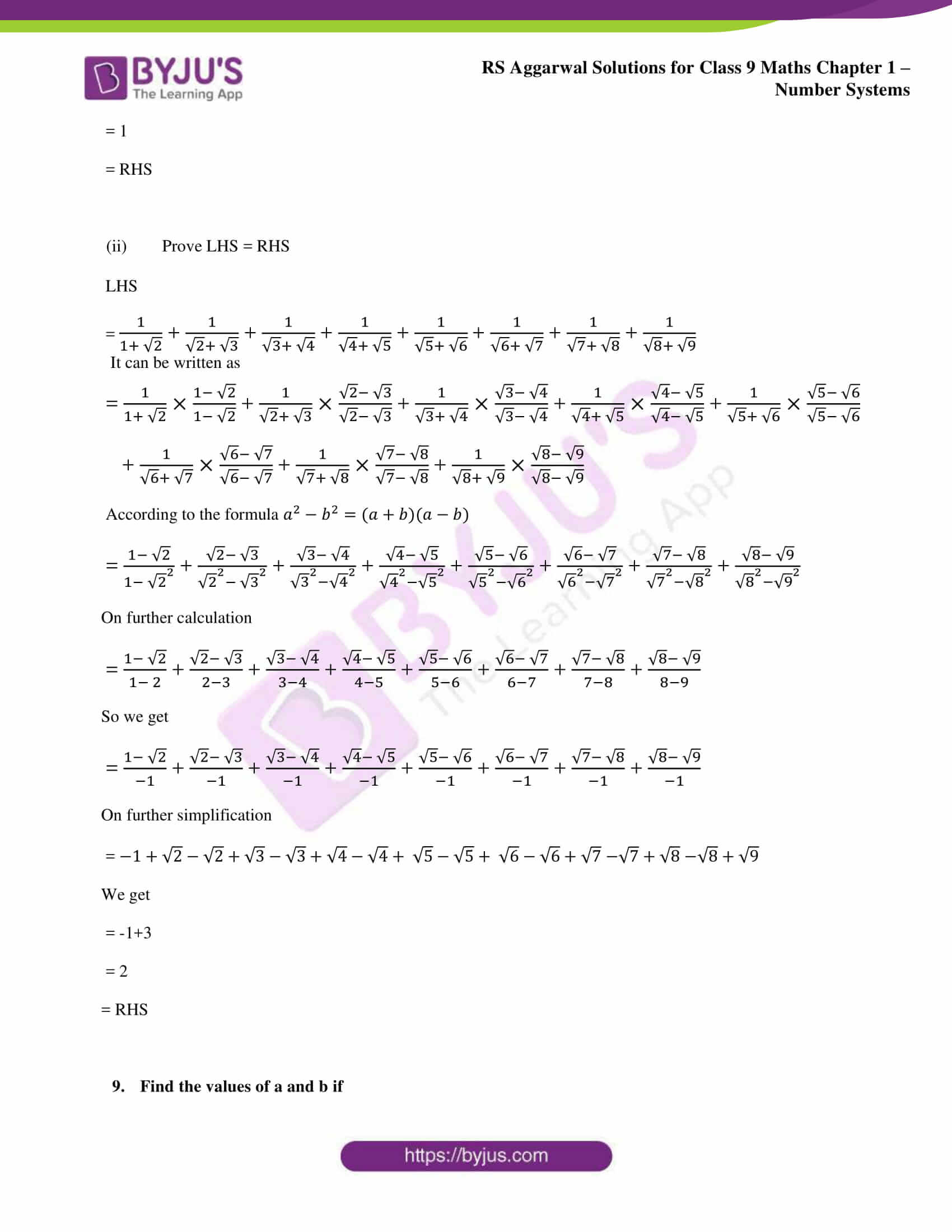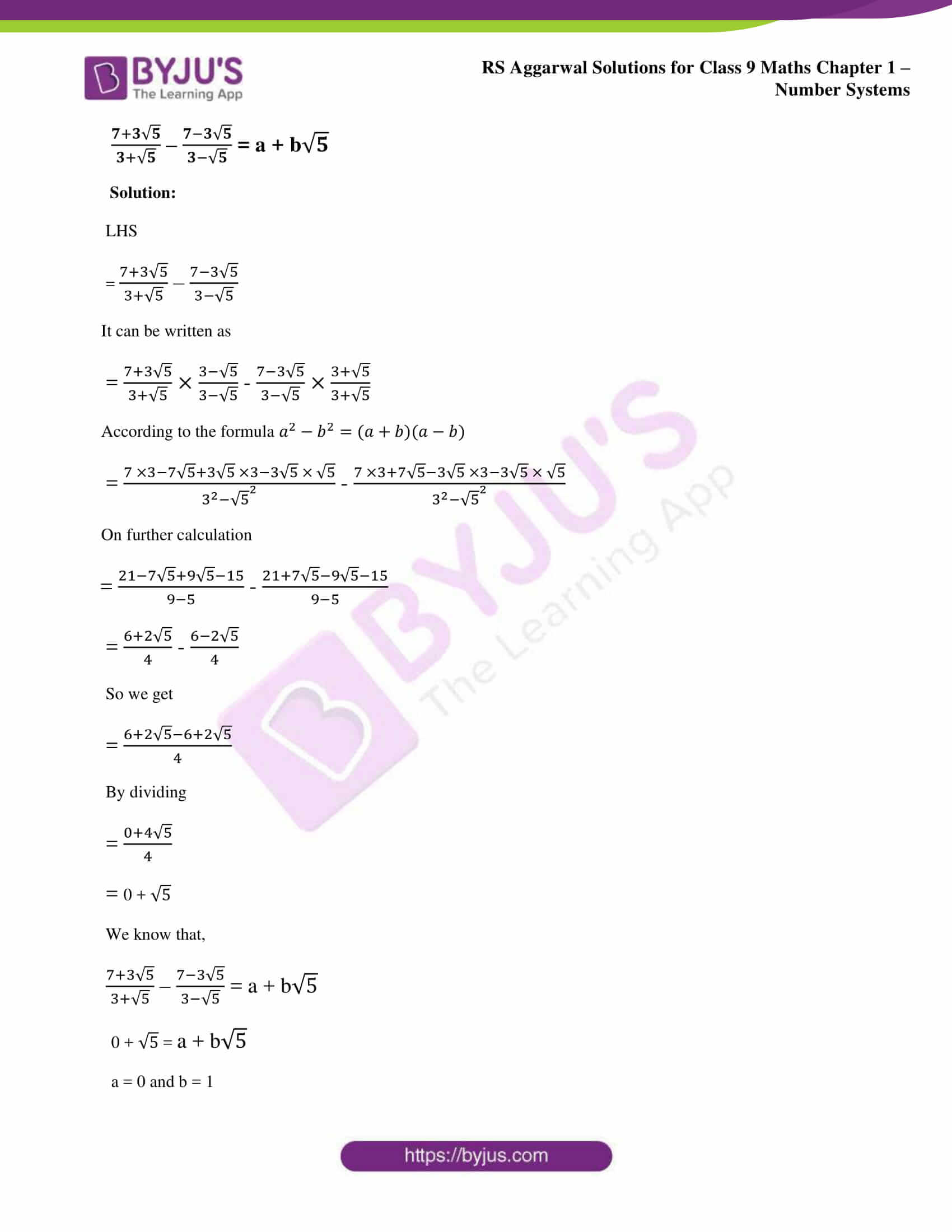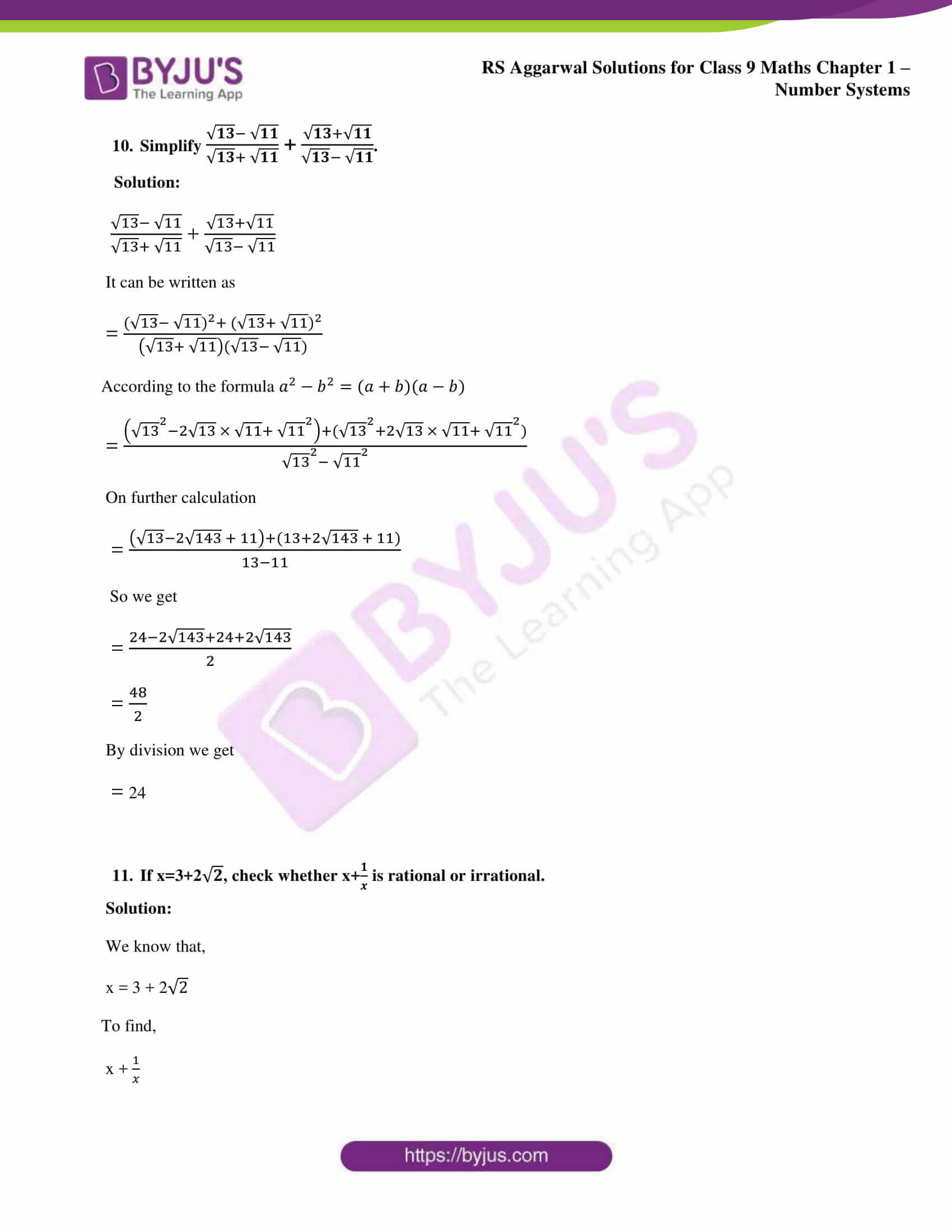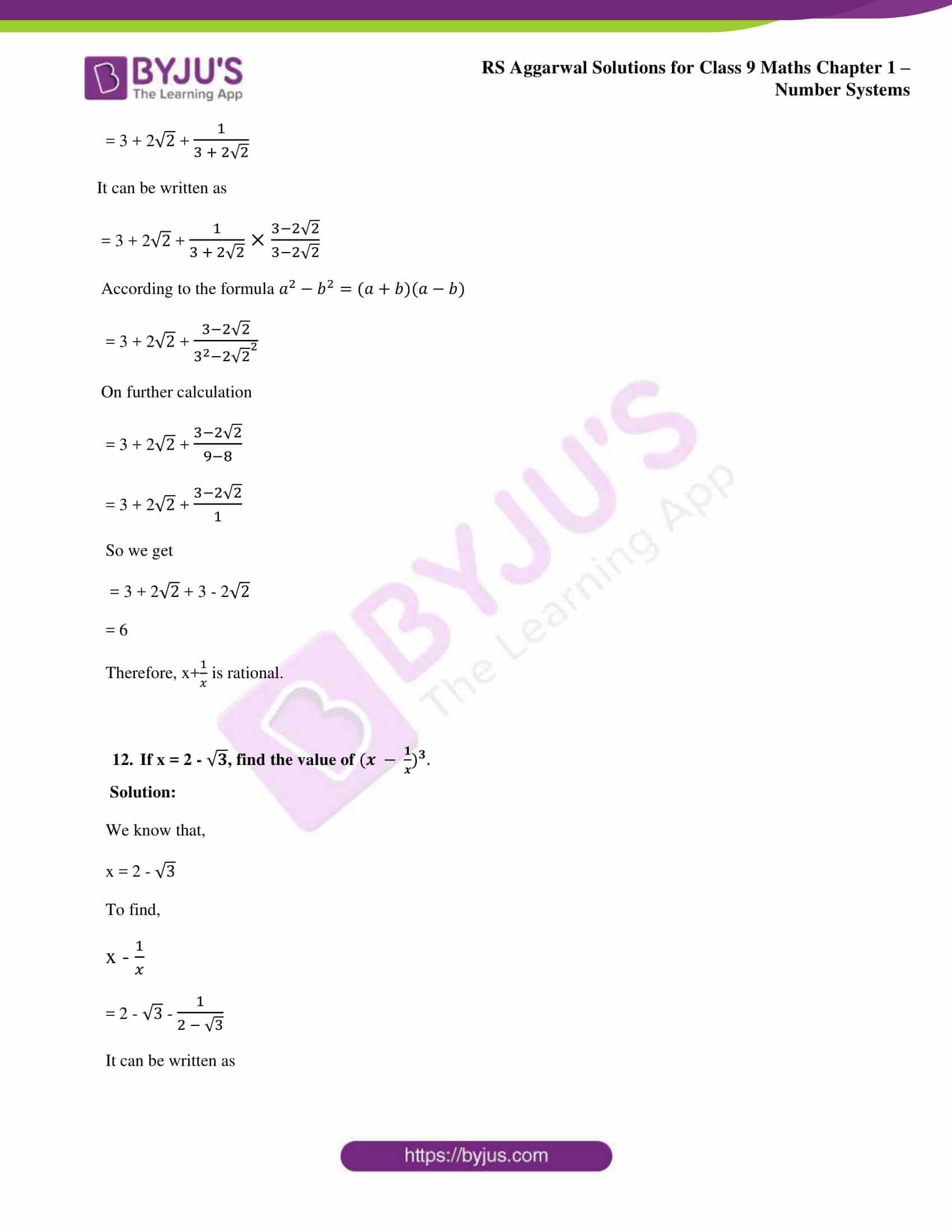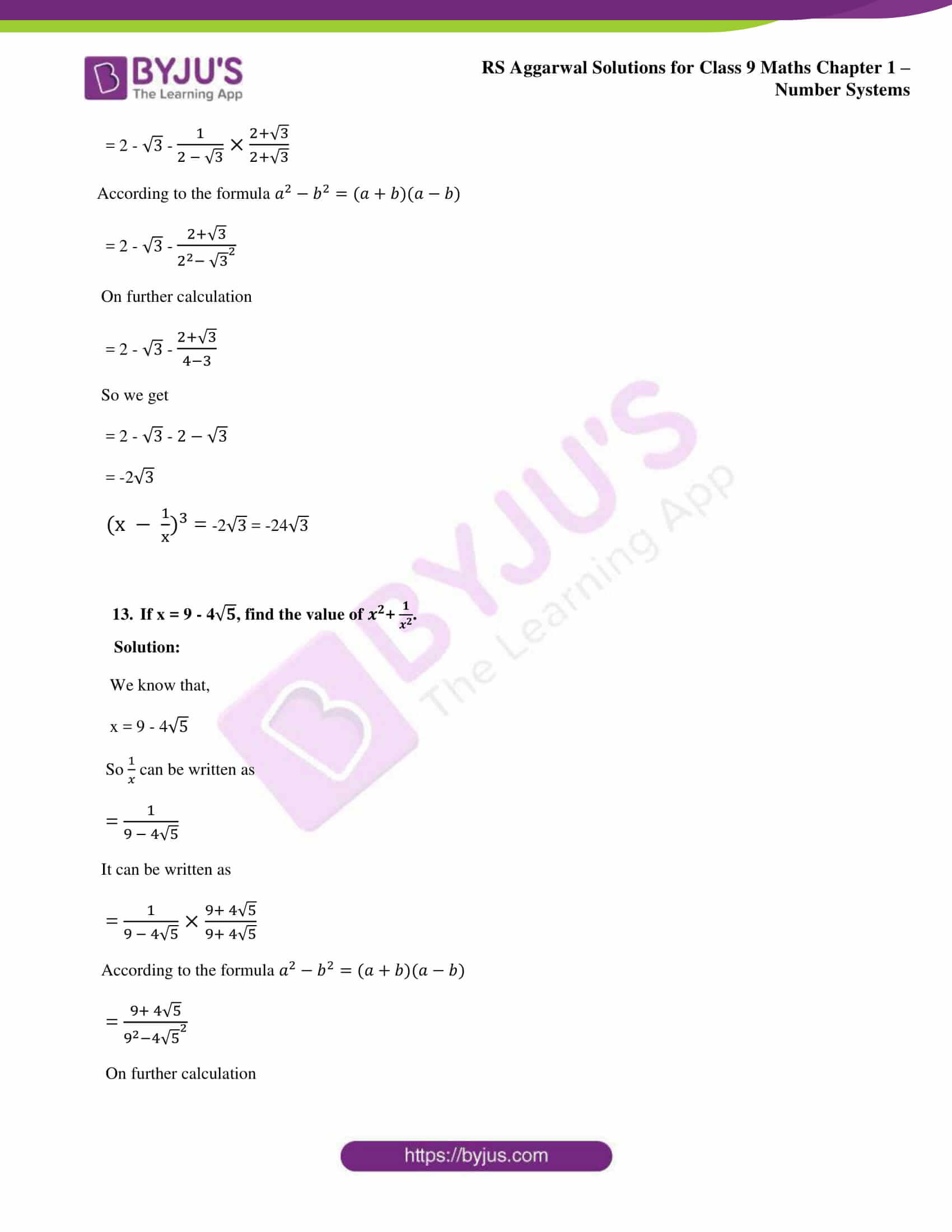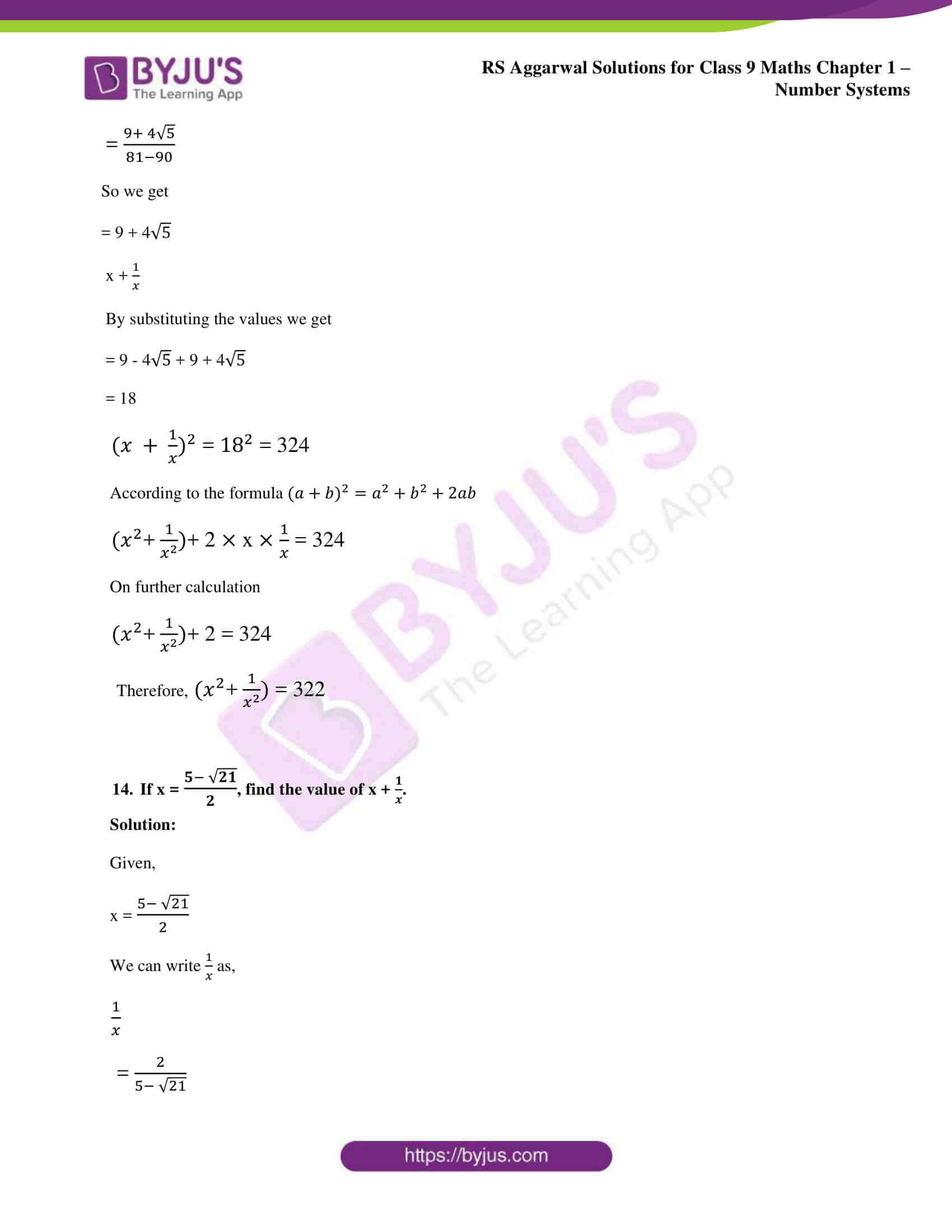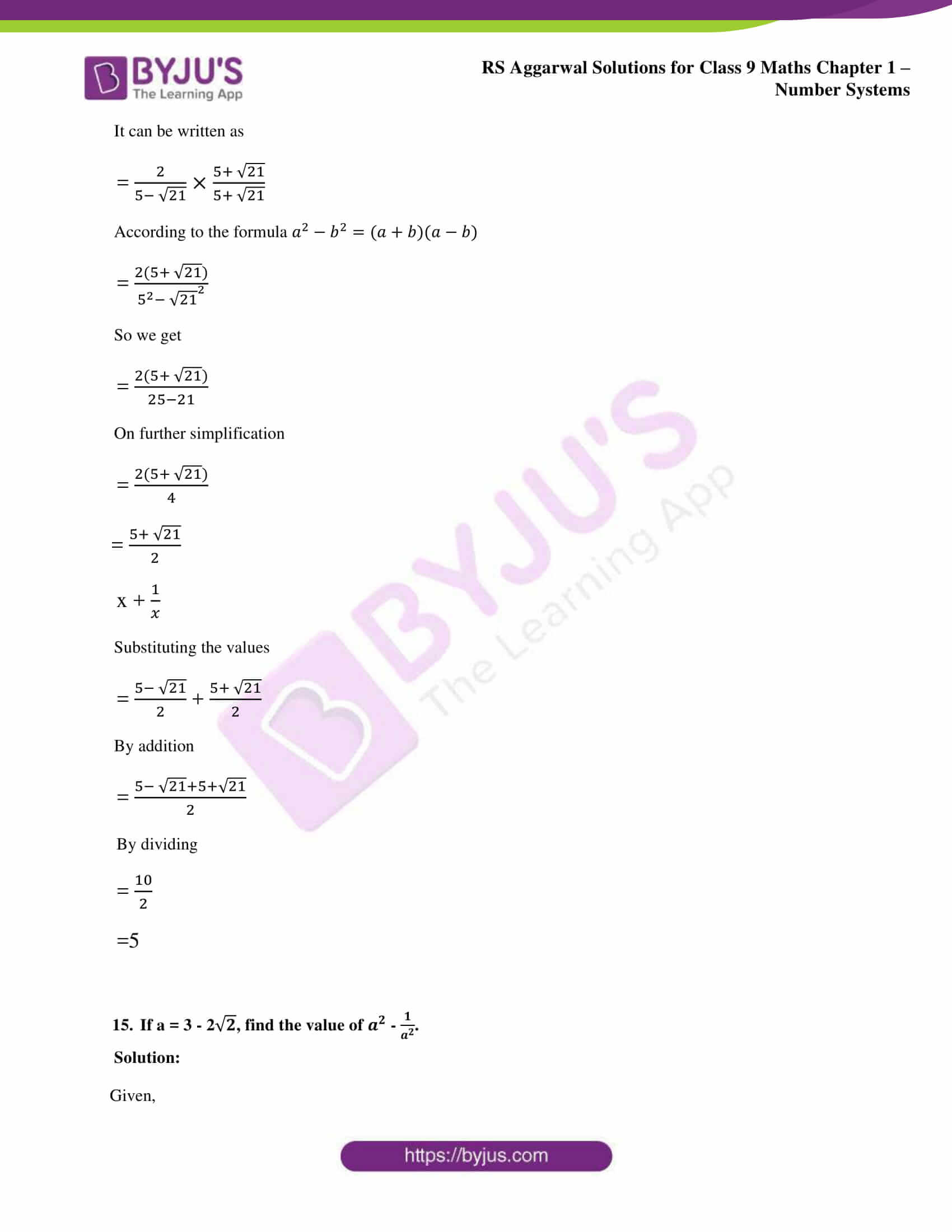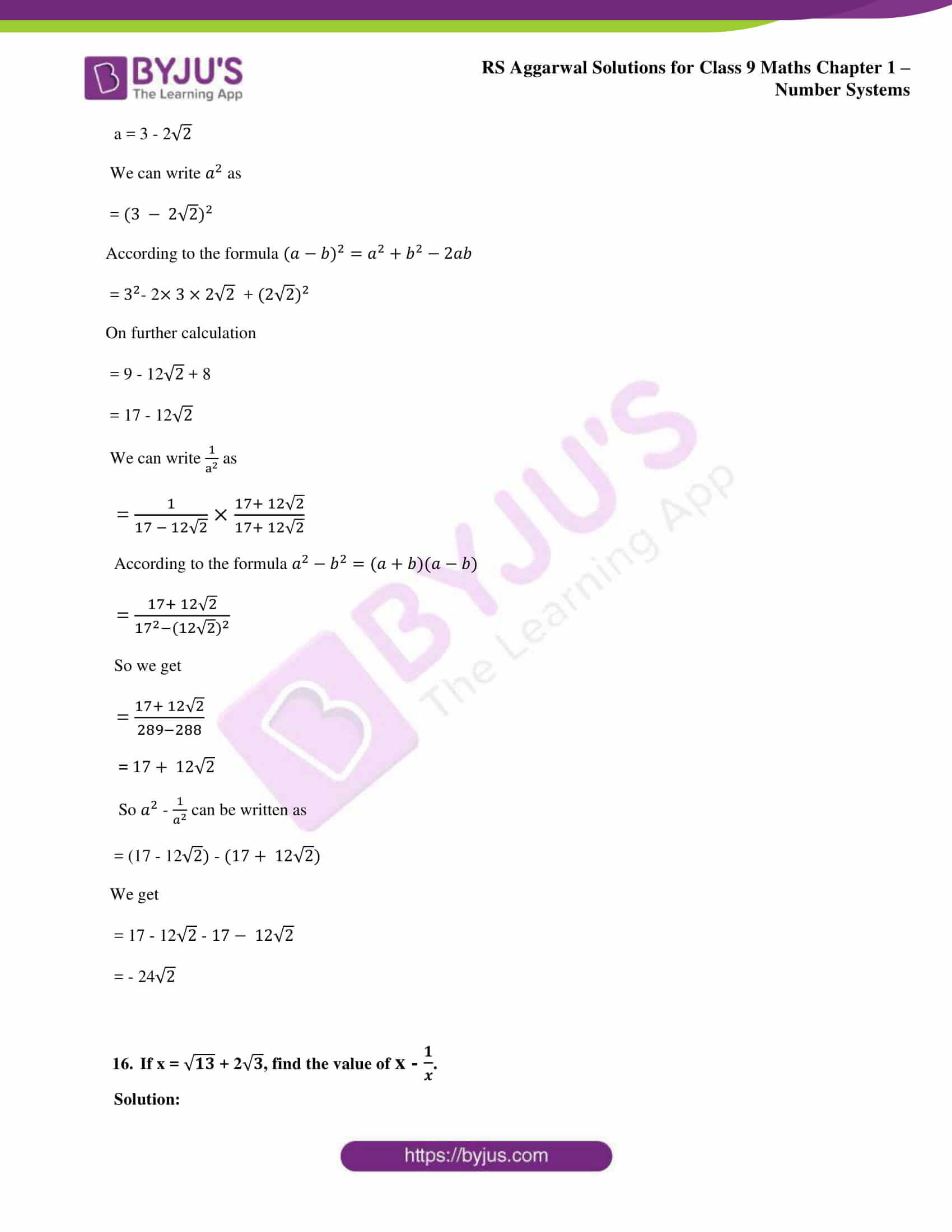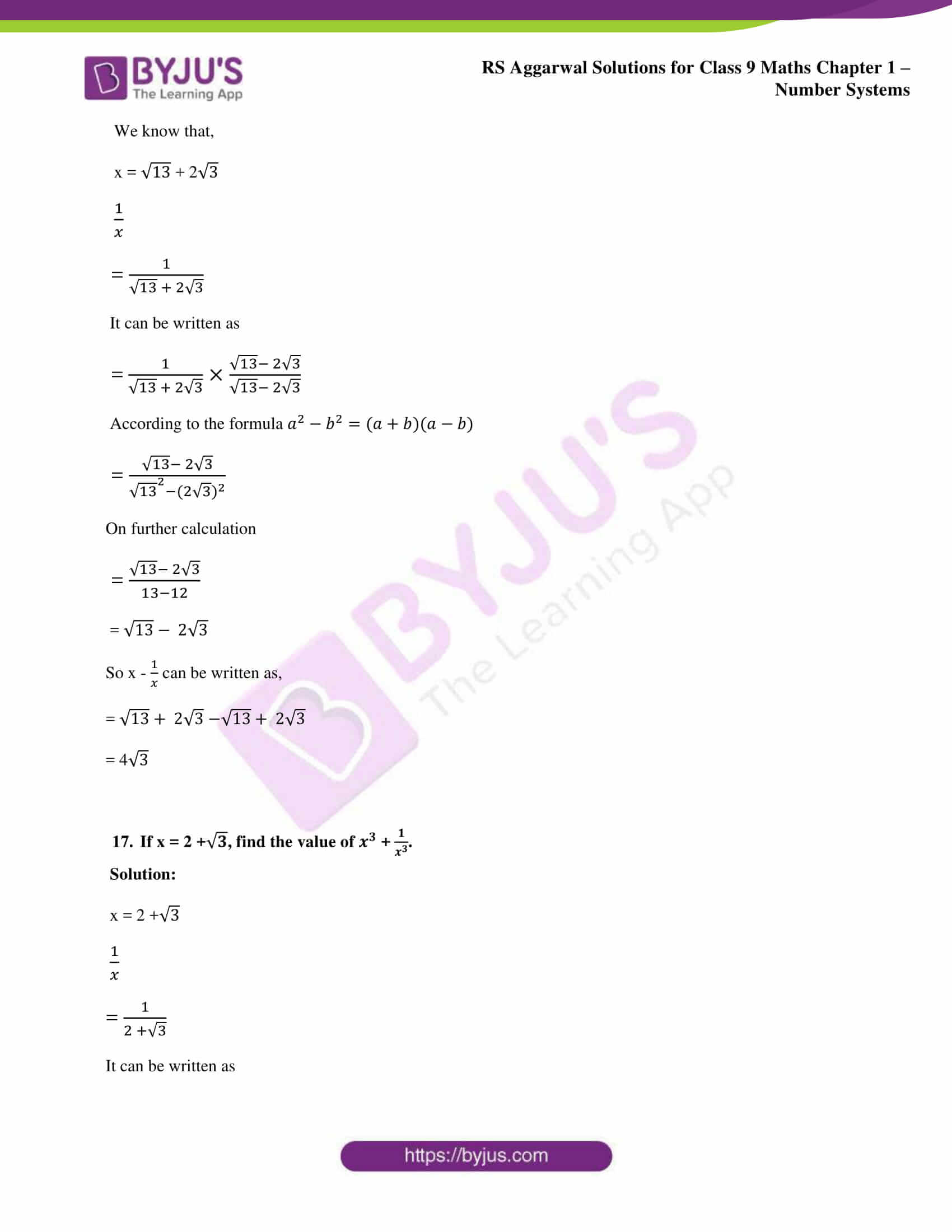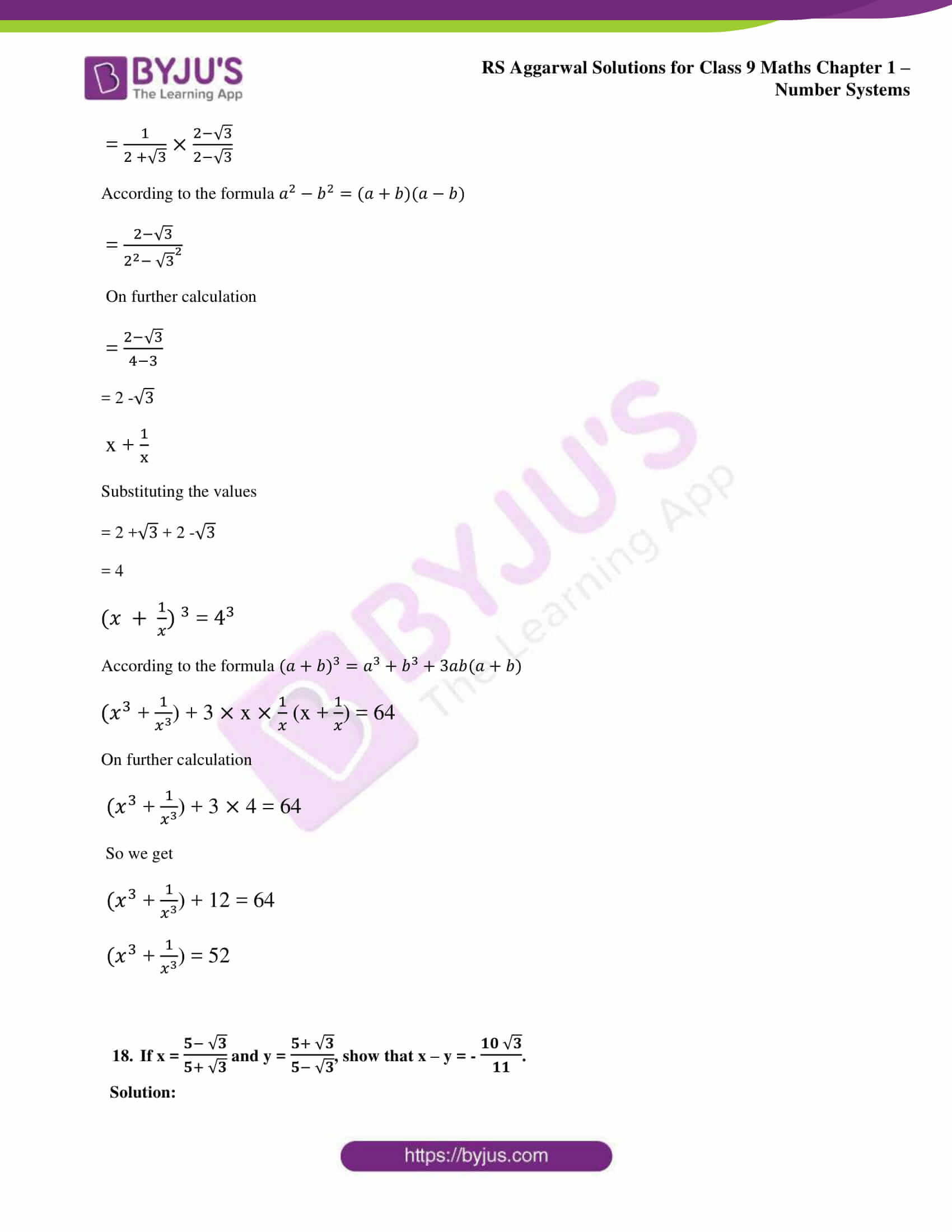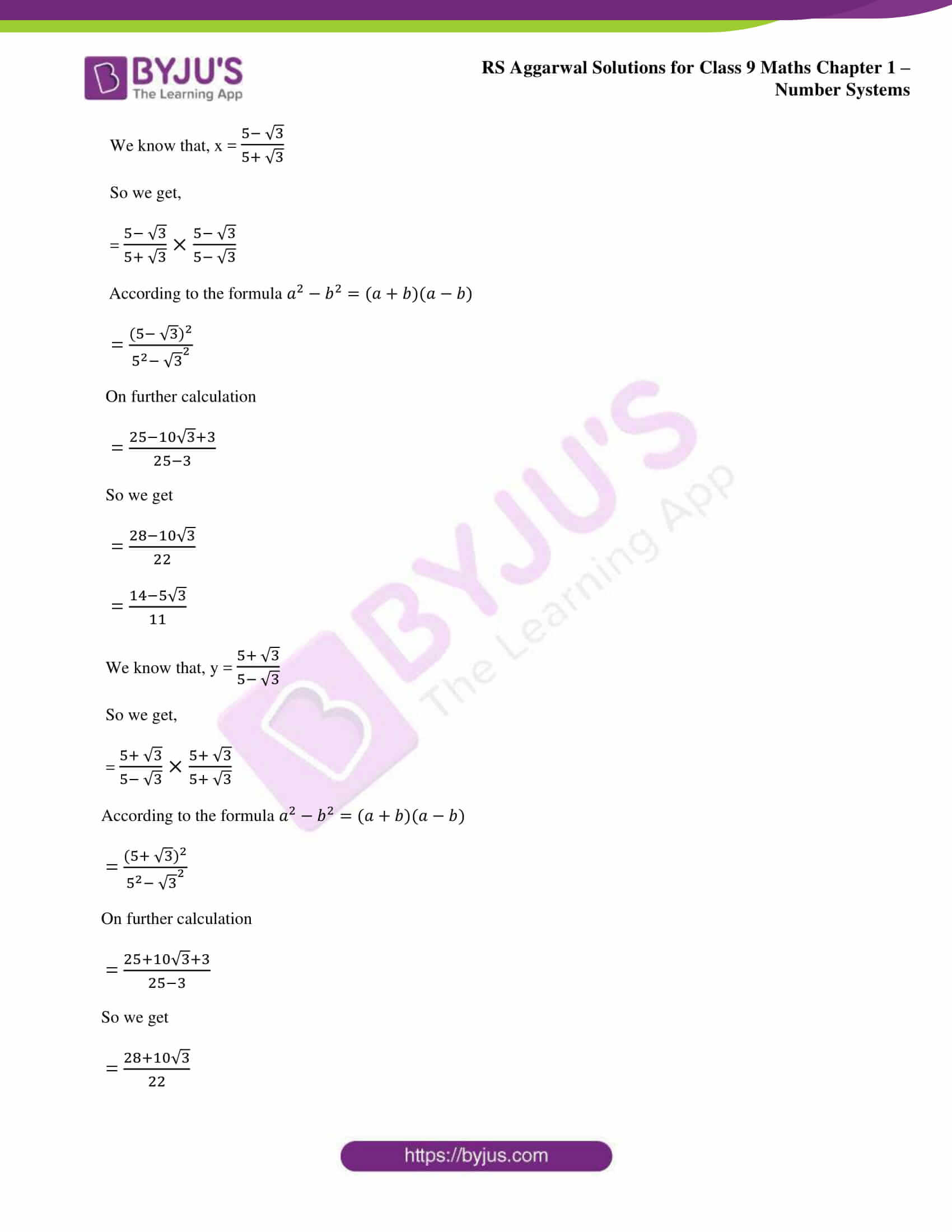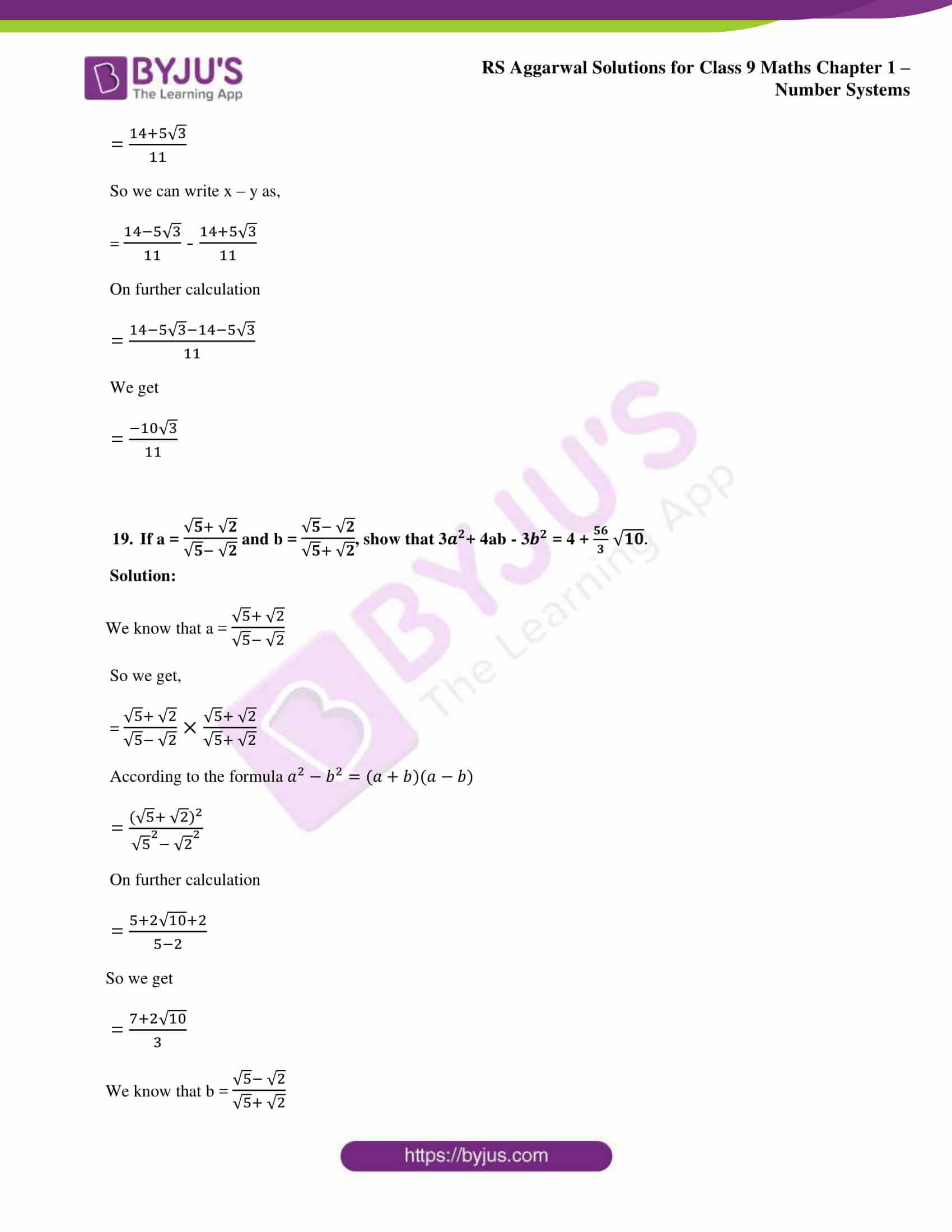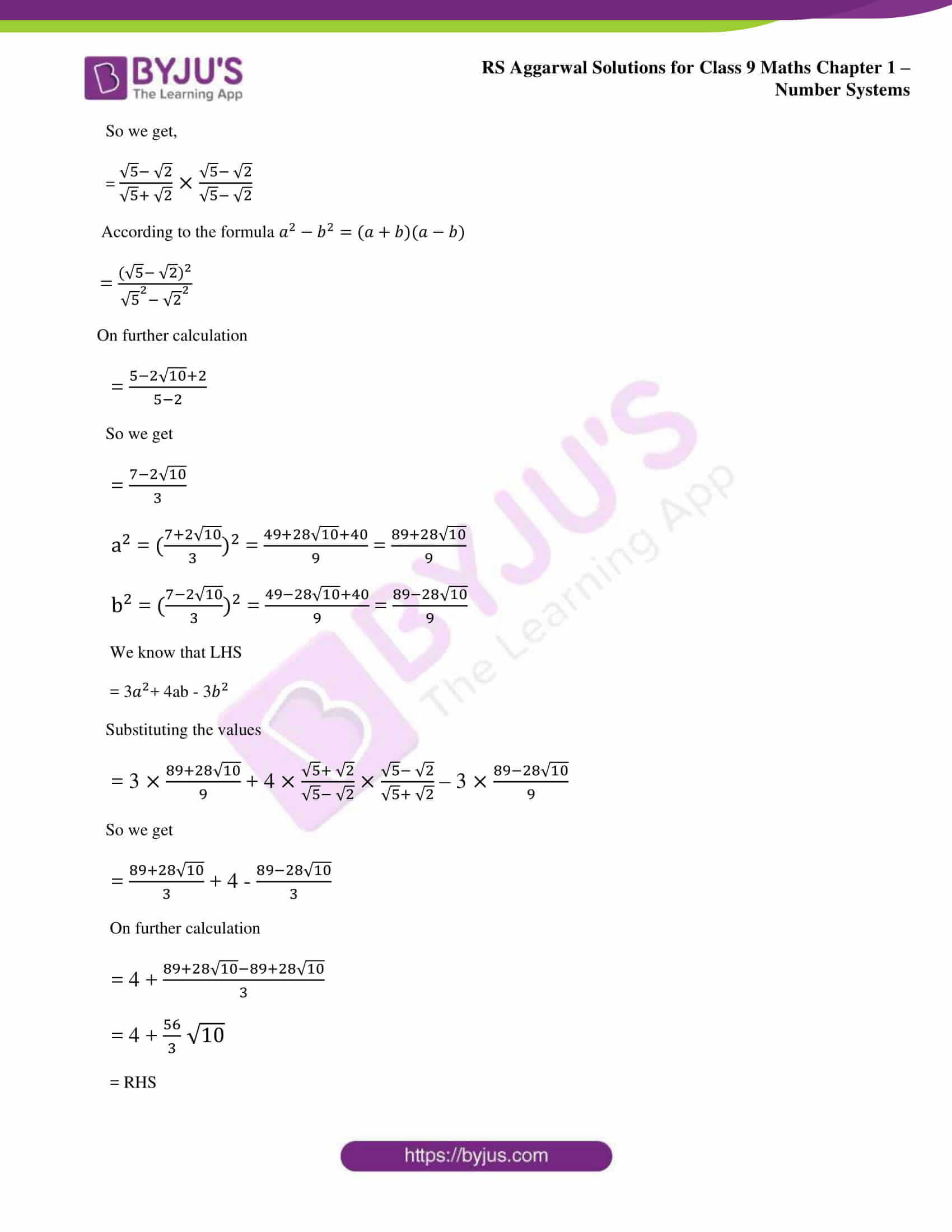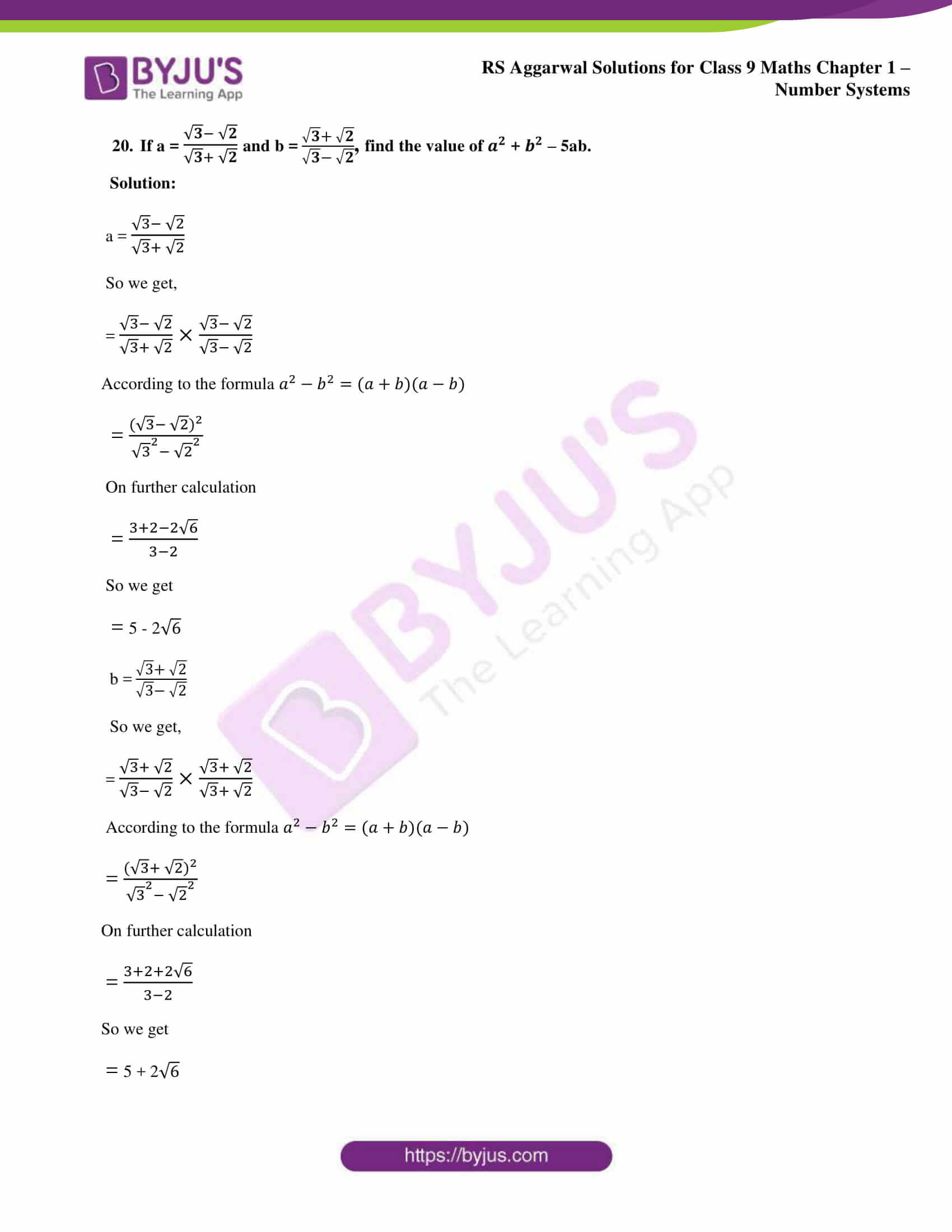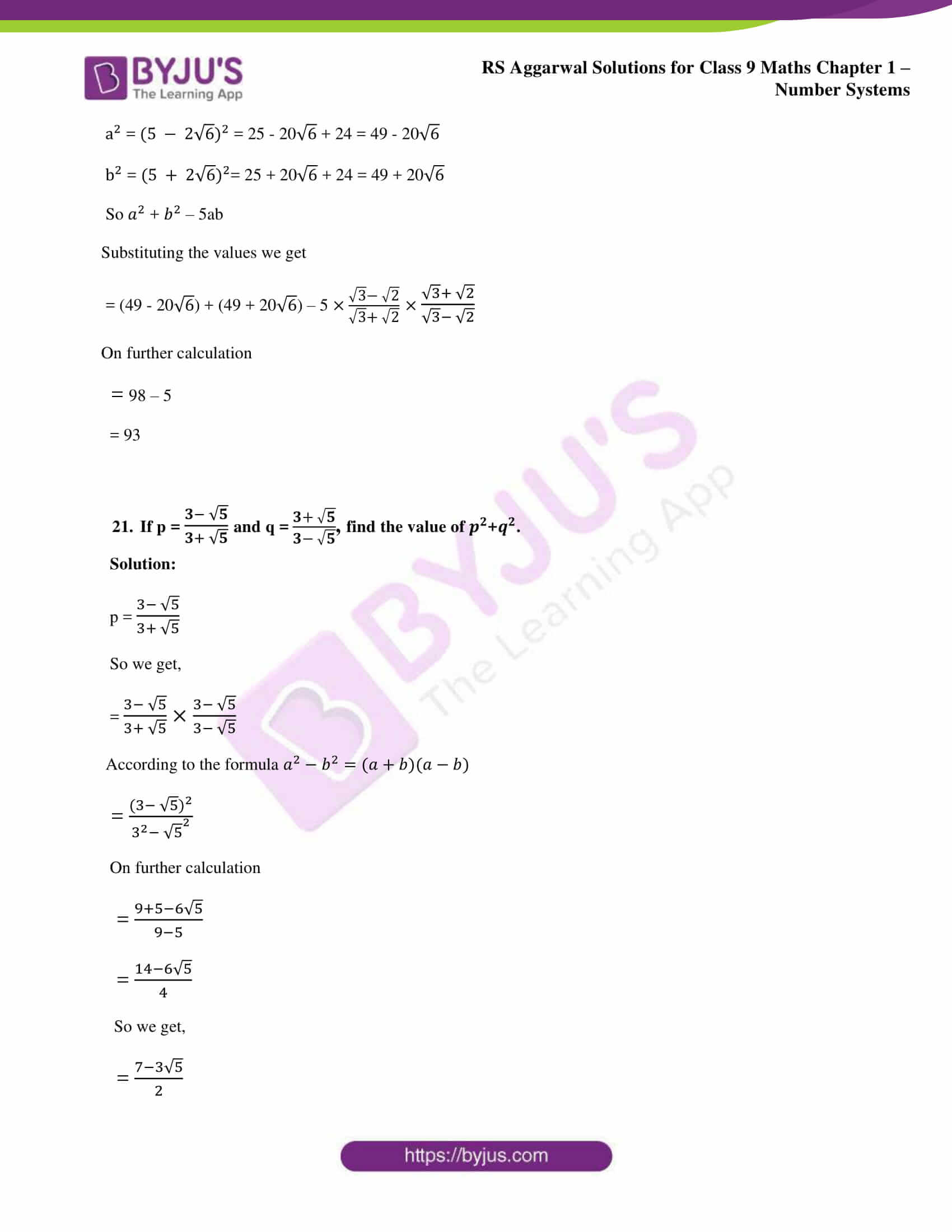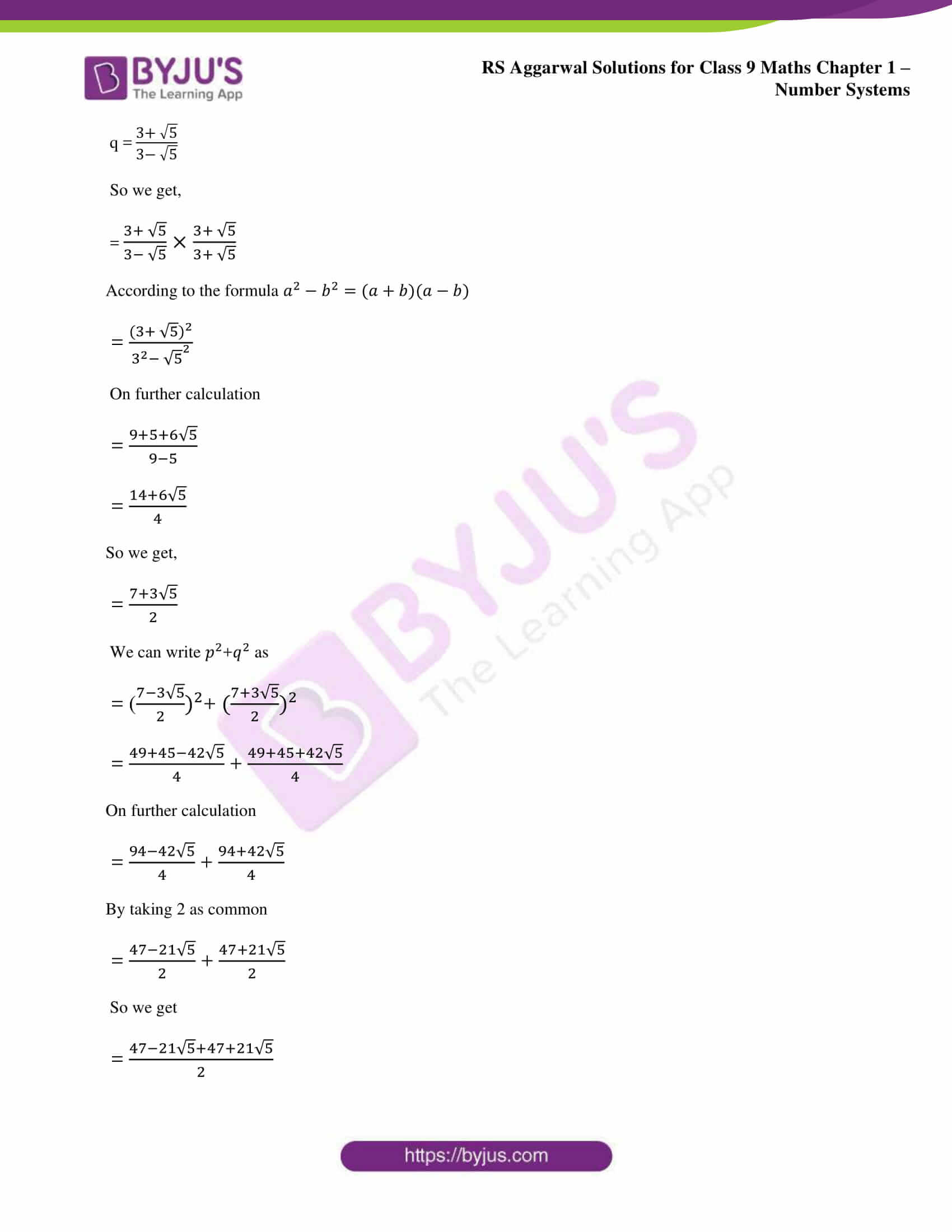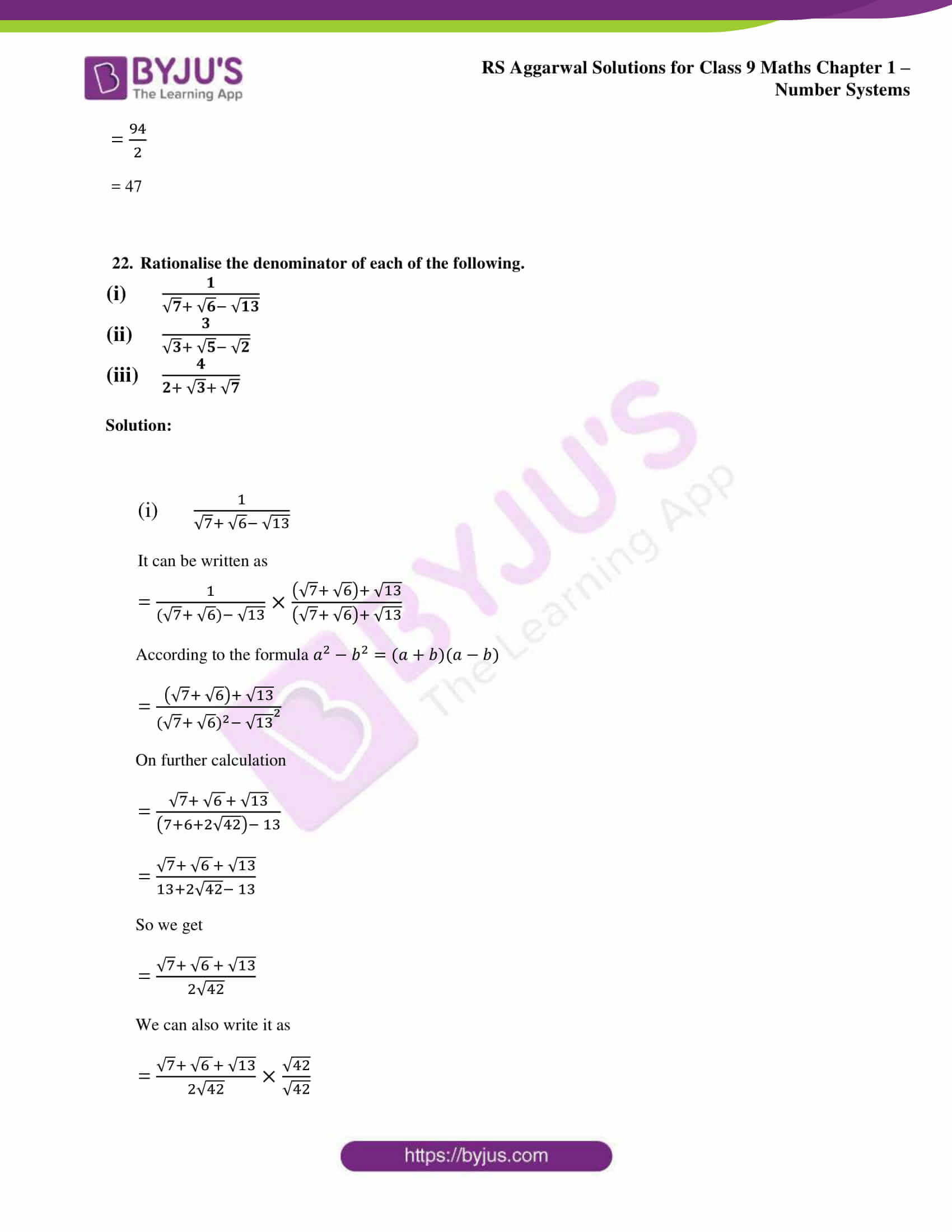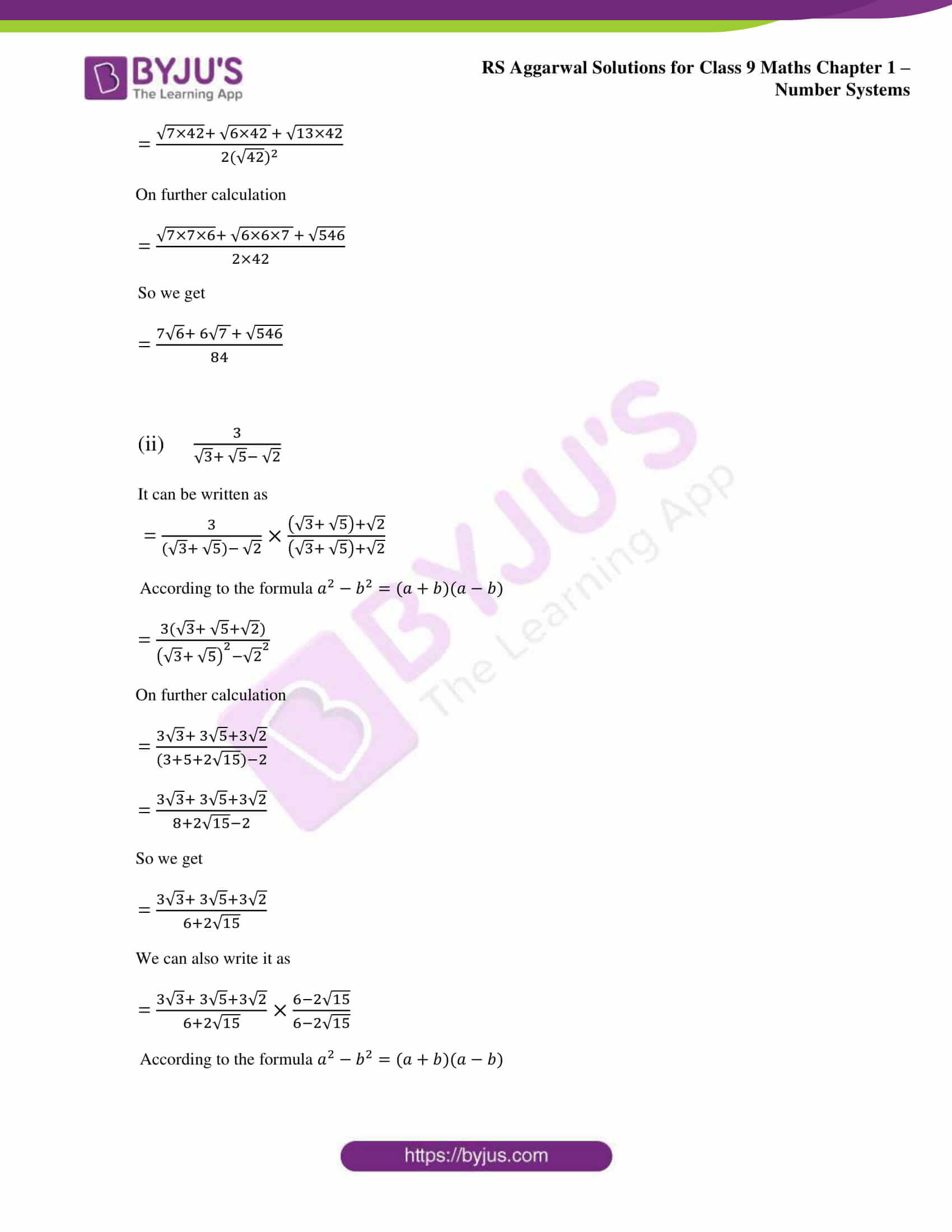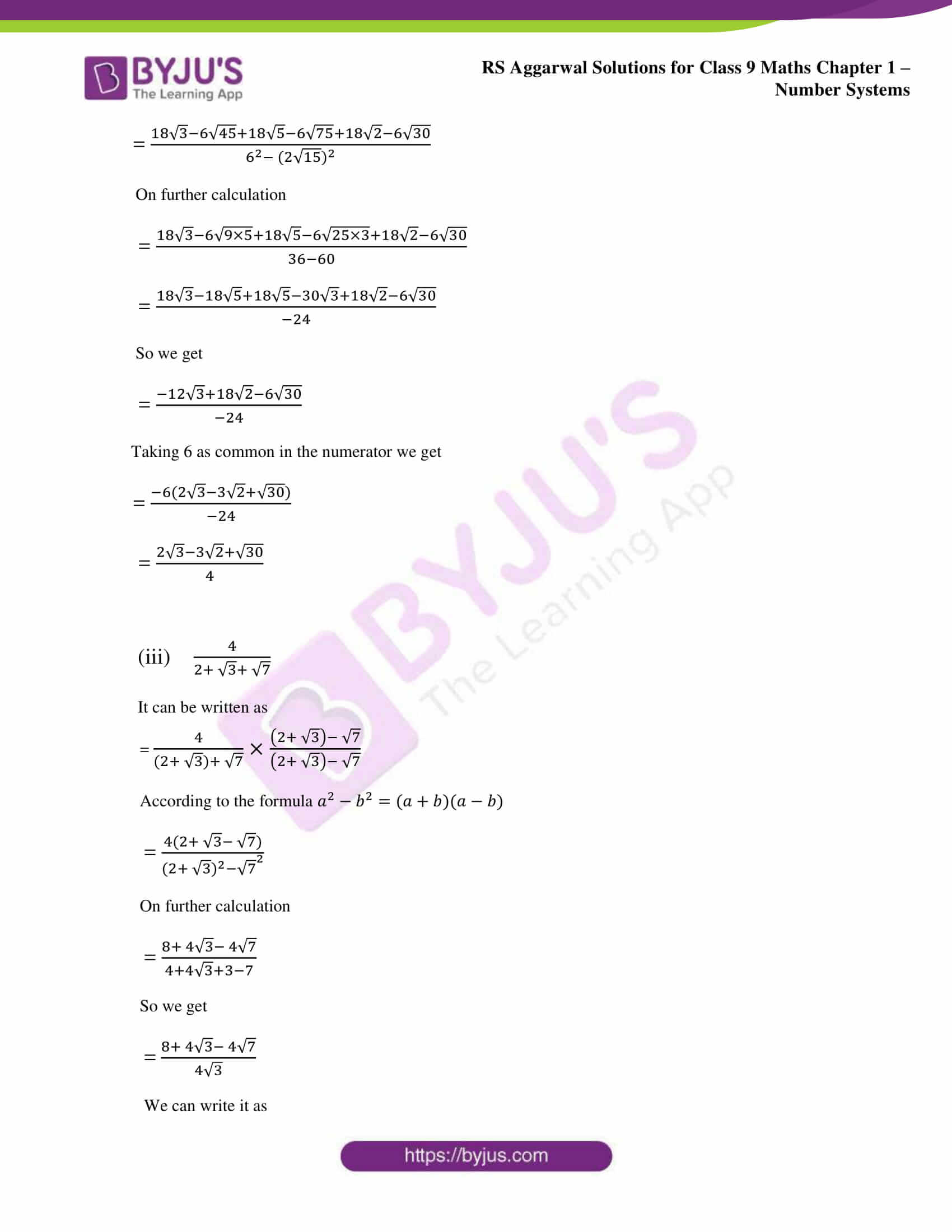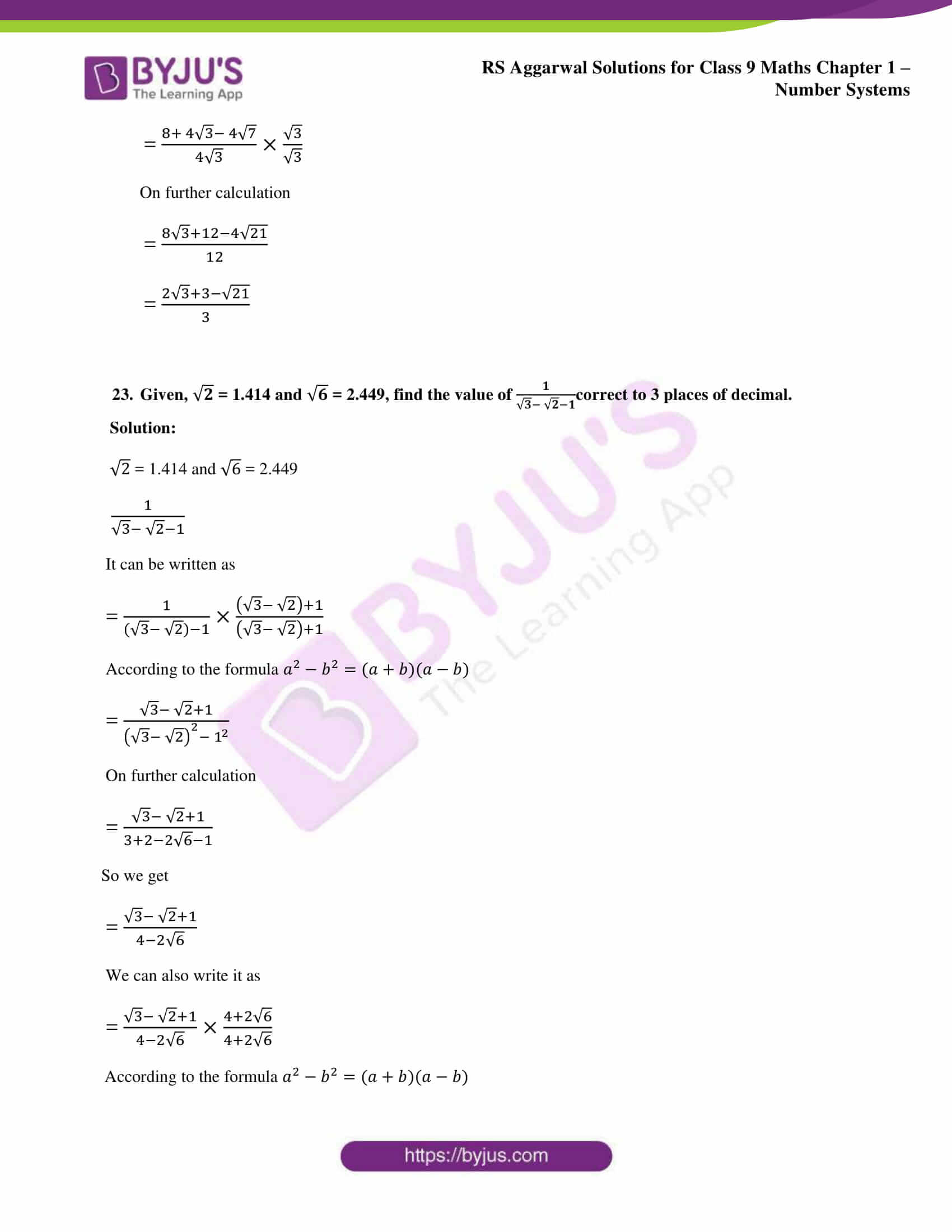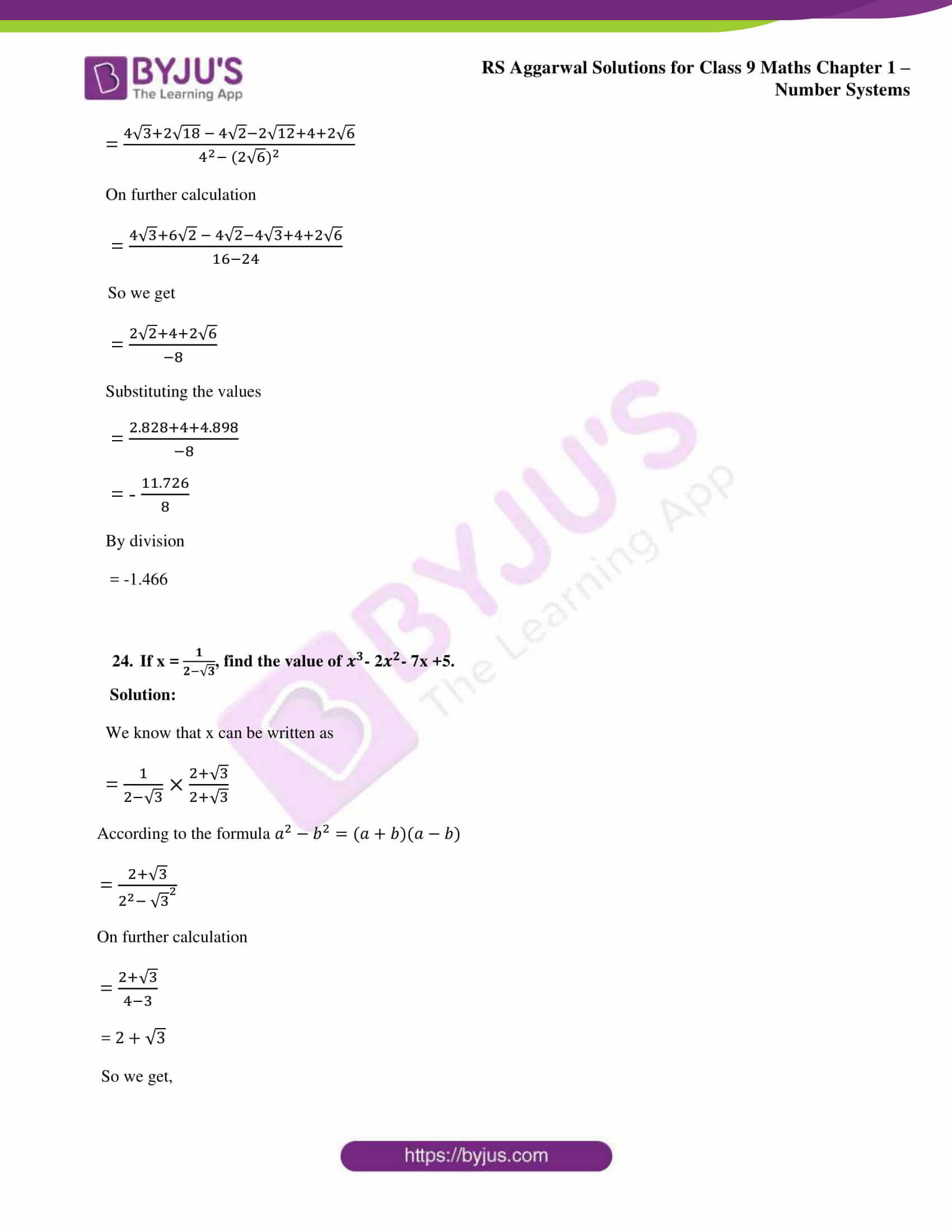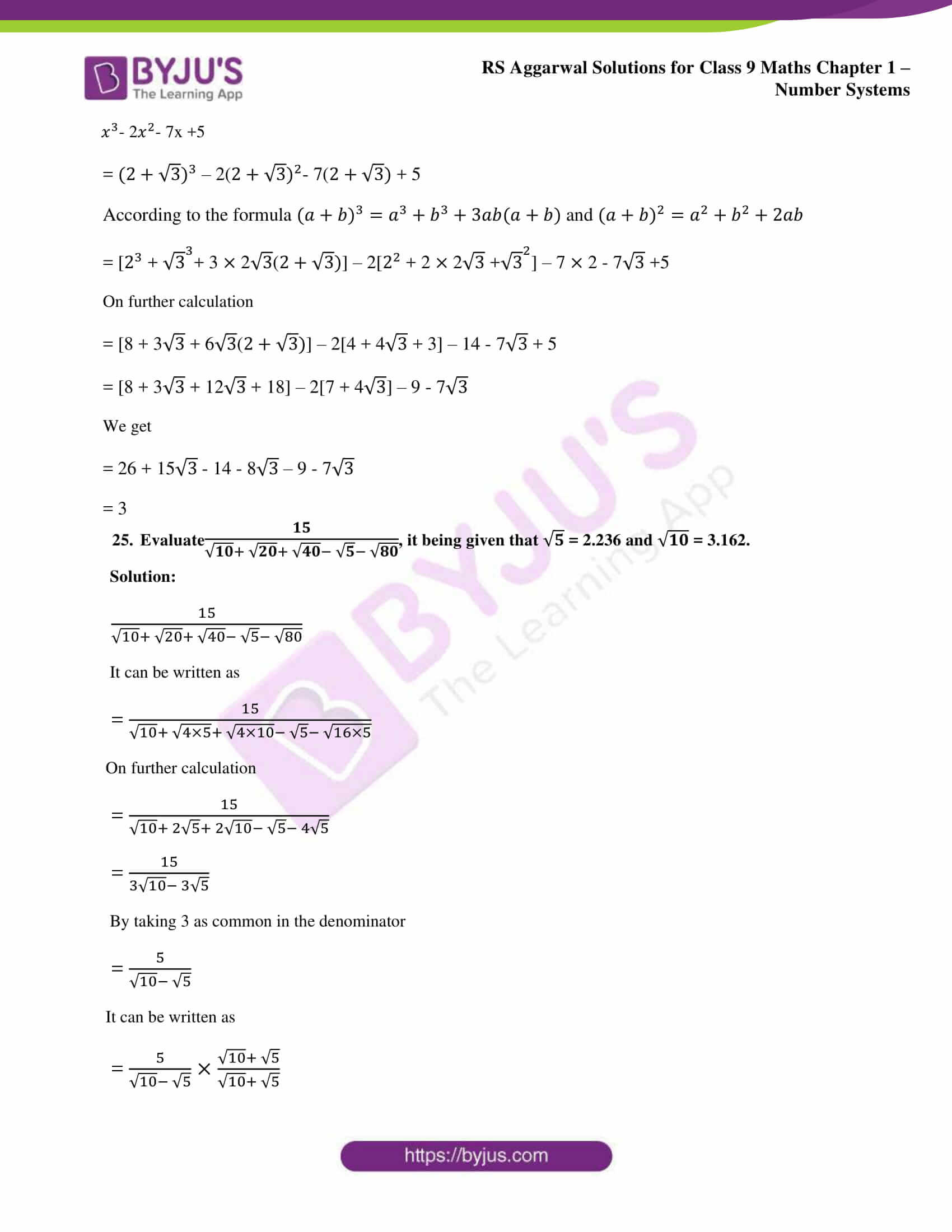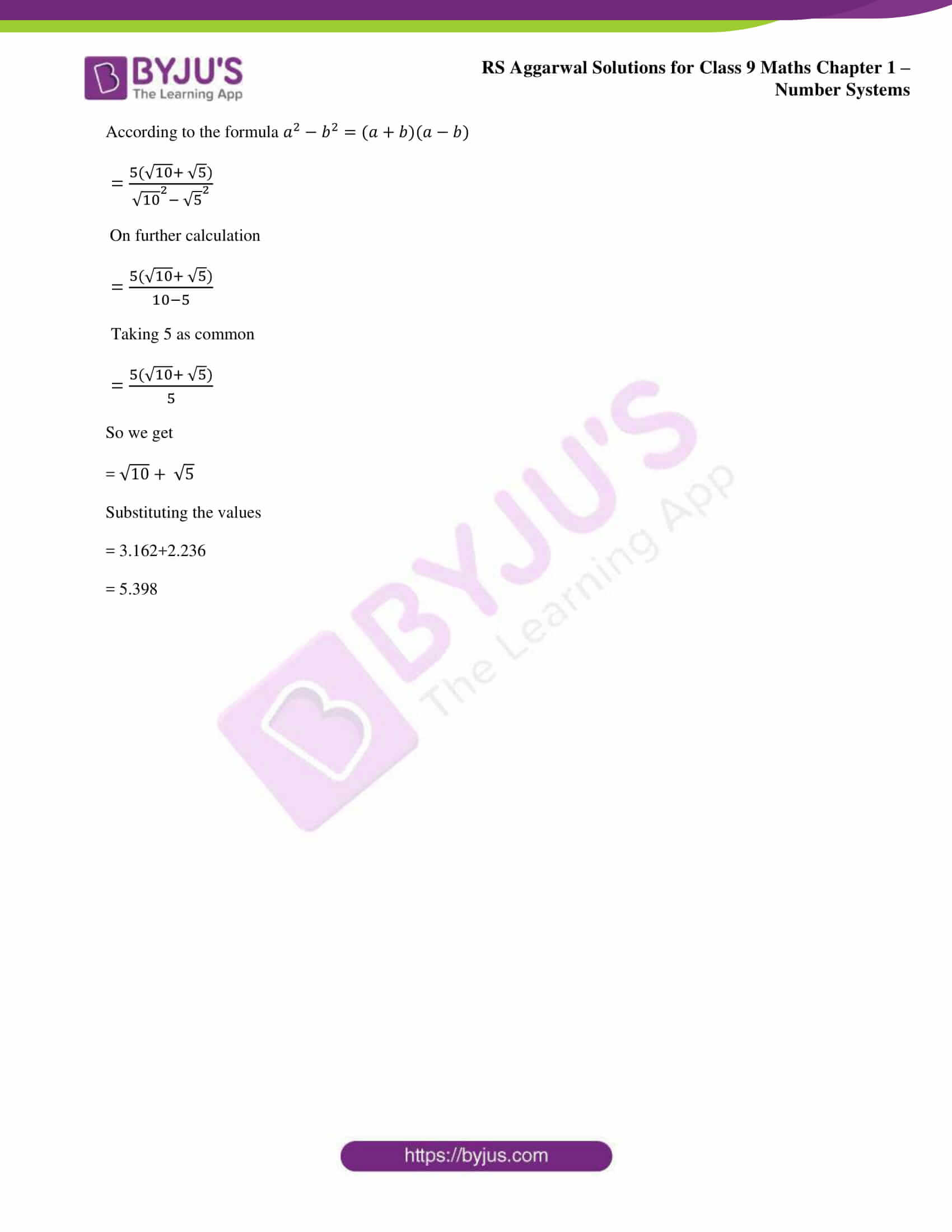### Access other exercise solutions of class 9 Maths Chapter 1: Number Systems

Exercise 1A Solutions 9 Questions

Exercise 1B Solutions 5 Questions

Exercise 1C Solutions 14 Questions

Exercise 1D Solutions 8 Questions

Exercise 1E Solutions 10 Questions

Exercise 1G Solutions 18 Questions

### RS Aggarwal Solutions Class 9 Maths Chapter 1 – Number Systems Exercise 1F

RS Aggarwal Solutions Class 9 Maths Chapter 1 Number Systems Exercise 1F explains students the various methods used in Rationalisation and ways to find Rationalising Factor.

### Key features of RS Aggarwal Solutions for Class 9 Maths Chapter 1: Number Systems Exercise 1F

• RS Aggarwal Solutions contains many number of problems to be solved in each exercise to help students build a positive approach towards exams.
• The students are provided with ample number of problems to solve with brief explanation and tips to improve speed.
• Solving problems according to RS Aggarwal textbook would help students improve their grip on concepts to appear for the exams.
• The formulas, theorems and important concepts are listed in each exercise before solving to make it easy for the students.